# JP2014523187A - Video intra-predictive encoding / decoding method and apparatus - Google Patents

## Info

Publication number
JP2014523187A
JP2014523187A JP2014518806A JP2014518806A JP2014523187A JP 2014523187 A JP2014523187 A JP 2014523187A JP 2014518806 A JP2014518806 A JP 2014518806A JP 2014518806 A JP2014518806 A JP 2014518806A JP 2014523187 A JP2014523187 A JP 2014523187A
Authority
JP
Japan
Prior art keywords
pixel
unit
current
coding
depth
Prior art date
Legal status (The legal status is an assumption and is not a legal conclusion. Google has not performed a legal analysis and makes no representation as to the accuracy of the status listed.)
Granted
Application number
JP2014518806A
Other languages
Japanese (ja)
Other versions
JP5956572B2 (en
Inventor
リー，テミー
チェン，ジアンル
Original Assignee
サムスン エレクトロニクス カンパニー リミテッド
Priority date (The priority date is an assumption and is not a legal conclusion. Google has not performed a legal analysis and makes no representation as to the accuracy of the date listed.)
Filing date
Publication date
Priority to US201161501969P priority Critical
Priority to US61/501,969 priority
Application filed by サムスン エレクトロニクス カンパニー リミテッド filed Critical サムスン エレクトロニクス カンパニー リミテッド
Priority to PCT/KR2012/005148 priority patent/WO2013002586A2/en
Publication of JP2014523187A publication Critical patent/JP2014523187A/en
Application granted granted Critical
Publication of JP5956572B2 publication Critical patent/JP5956572B2/en
Application status is Active legal-status Critical
Anticipated expiration legal-status Critical

## Images

•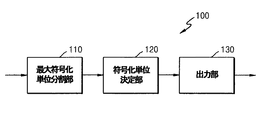•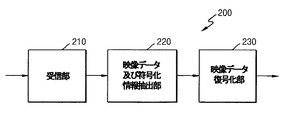•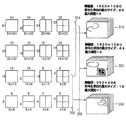•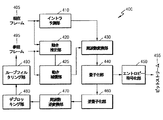•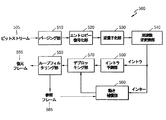•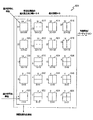•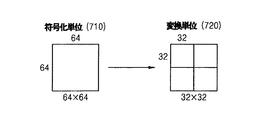••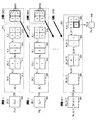•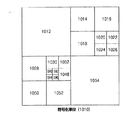•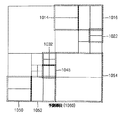•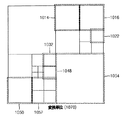•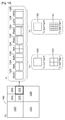•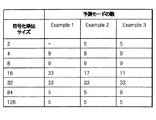•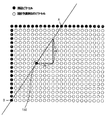•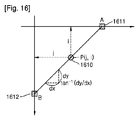•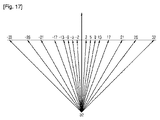••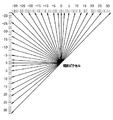•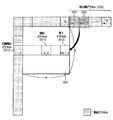•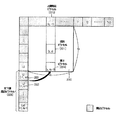•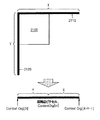•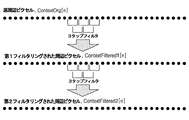•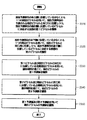## Classifications

• HELECTRICITY
• H04ELECTRIC COMMUNICATION TECHNIQUE
• H04NPICTORIAL COMMUNICATION, e.g. TELEVISION
• H04N19/00Methods or arrangements for coding, decoding, compressing or decompressing digital video signals
• H04N19/50Methods or arrangements for coding, decoding, compressing or decompressing digital video signals using predictive coding
• H04N19/503Methods or arrangements for coding, decoding, compressing or decompressing digital video signals using predictive coding involving temporal prediction
• H04N19/51Motion estimation or motion compensation
• H04N19/513Processing of motion vectors
• H04N19/517Processing of motion vectors by encoding
• H04N19/52Processing of motion vectors by encoding by predictive encoding
• HELECTRICITY
• H04ELECTRIC COMMUNICATION TECHNIQUE
• H04NPICTORIAL COMMUNICATION, e.g. TELEVISION
• H04N19/00Methods or arrangements for coding, decoding, compressing or decompressing digital video signals
• H04N19/10Methods or arrangements for coding, decoding, compressing or decompressing digital video signals using adaptive coding
• H04N19/102Methods or arrangements for coding, decoding, compressing or decompressing digital video signals using adaptive coding characterised by the element, parameter or selection affected or controlled by the adaptive coding
• H04N19/13Adaptive entropy coding, e.g. adaptive variable length coding [AVLC] or context adaptive binary arithmetic coding [CABAC]
• HELECTRICITY
• H04ELECTRIC COMMUNICATION TECHNIQUE
• H04NPICTORIAL COMMUNICATION, e.g. TELEVISION
• H04N19/00Methods or arrangements for coding, decoding, compressing or decompressing digital video signals
• H04N19/50Methods or arrangements for coding, decoding, compressing or decompressing digital video signals using predictive coding
• H04N19/503Methods or arrangements for coding, decoding, compressing or decompressing digital video signals using predictive coding involving temporal prediction
• H04N19/51Motion estimation or motion compensation
• HELECTRICITY
• H04ELECTRIC COMMUNICATION TECHNIQUE
• H04NPICTORIAL COMMUNICATION, e.g. TELEVISION
• H04N19/00Methods or arrangements for coding, decoding, compressing or decompressing digital video signals
• H04N19/50Methods or arrangements for coding, decoding, compressing or decompressing digital video signals using predictive coding
• H04N19/593Methods or arrangements for coding, decoding, compressing or decompressing digital video signals using predictive coding involving spatial prediction techniques
• HELECTRICITY
• H04ELECTRIC COMMUNICATION TECHNIQUE
• H04NPICTORIAL COMMUNICATION, e.g. TELEVISION
• H04N19/00Methods or arrangements for coding, decoding, compressing or decompressing digital video signals
• H04N19/60Methods or arrangements for coding, decoding, compressing or decompressing digital video signals using transform coding
• H04N19/61Methods or arrangements for coding, decoding, compressing or decompressing digital video signals using transform coding in combination with predictive coding
• HELECTRICITY
• H04ELECTRIC COMMUNICATION TECHNIQUE
• H04NPICTORIAL COMMUNICATION, e.g. TELEVISION
• H04N19/00Methods or arrangements for coding, decoding, compressing or decompressing digital video signals
• H04N19/80Details of filtering operations specially adapted for video compression, e.g. for pixel interpolation
• H04N19/82Details of filtering operations specially adapted for video compression, e.g. for pixel interpolation involving filtering within a prediction loop
• HELECTRICITY
• H04ELECTRIC COMMUNICATION TECHNIQUE
• H04NPICTORIAL COMMUNICATION, e.g. TELEVISION
• H04N19/00Methods or arrangements for coding, decoding, compressing or decompressing digital video signals
• H04N19/90Methods or arrangements for coding, decoding, compressing or decompressing digital video signals using coding techniques not provided for in groups H04N19/10-H04N19/85, e.g. fractals
• H04N19/91Entropy coding, e.g. variable length coding [VLC] or arithmetic coding
• HELECTRICITY
• H04ELECTRIC COMMUNICATION TECHNIQUE
• H04NPICTORIAL COMMUNICATION, e.g. TELEVISION
• H04N19/00Methods or arrangements for coding, decoding, compressing or decompressing digital video signals
• H04N19/90Methods or arrangements for coding, decoding, compressing or decompressing digital video signals using coding techniques not provided for in groups H04N19/10-H04N19/85, e.g. fractals
• H04N19/96Tree coding, e.g. quad-tree coding

## Abstract

A video intra prediction method and apparatus for generating prediction values through horizontal and vertical linear interpolation for a current prediction unit are disclosed. The method for intra prediction of a video according to the present invention generates a virtual first pixel and a second pixel using at least one neighboring pixel located at an upper right side and a lower left side of a current prediction unit, and the first pixel and the second pixel are generated. The first predicted value of the current pixel is obtained through linear interpolation using the left peripheral pixel located in the same row as the current pixel, and the upper peripheral pixel located in the same column as the second pixel and the current pixel is used. A second prediction value of the current pixel is obtained through linear interpolation, and a prediction value of the current pixel is obtained using the first prediction value and the second prediction value.

## Description

The present invention relates to video encoding and decoding, and more specifically, a video intra prediction encoding / decoding method that improves video compression efficiency using various directions and new intra prediction modes. And an apparatus.

MPEG-1, MPEG-2, MPEG-4, H.264. In a video compression scheme such as H.264 / MPEG-4 AVC (Advanced Video Coding), one picture is divided into macroblocks in order to encode the video. Then, after encoding each macroblock in all encoding modes that can be used in inter prediction and intra prediction, the bit rate for encoding the macroblock, the original macroblock, and the decoded macroblock One encoding mode is selected according to the degree of distortion, and the macroblock is encoded.

With the development and popularization of hardware that can play and store high-resolution or high-quality video content, the need for video codecs that effectively encode or decode high-resolution or high-quality video content is increasing. According to the existing video codec, the video is encoded by a limited prediction mode based on a macroblock of a predetermined size.

A technical problem to be solved by the present invention is to improve coding efficiency according to video characteristics through a new intra prediction method using neighboring pixels of a current prediction unit.

The present invention also provides a new type of intra prediction mode using neighboring pixels of the current prediction unit.

The method for intra prediction of a video according to an embodiment of the present invention uses at least one neighboring pixel located on the upper right side of the current prediction unit and is located in the same row as the predicted current pixel of the current prediction unit. Meanwhile, obtaining a virtual first pixel corresponding to a pixel located on the rightmost side of the current prediction unit, and using at least one neighboring pixel located on the lower left side of the current prediction unit Obtaining a virtual second pixel corresponding to a pixel located at the bottom of the current prediction unit while being in the same column as the current pixel; in the same row as the first pixel and the current pixel; Obtaining a first predicted value of the current pixel through linear interpolation using a left peripheral pixel that is positioned; and the same as the second pixel and the current pixel. Obtaining a second predicted value of the current pixel through linear interpolation using upper peripheral pixels located in a row, and using the first predicted value and the second predicted value, the predicted value of the current pixel And obtaining a stage.

An intra prediction apparatus for a video according to an embodiment of the present invention is located in the same row as a predicted current pixel of the current prediction unit using at least one neighboring pixel located on the upper right side of the current prediction unit. Meanwhile, a virtual first pixel corresponding to a pixel located on the rightmost side of the current prediction unit is obtained, and at least one neighboring pixel located on the lower left side of the current prediction unit is used to Obtaining a virtual second pixel corresponding to the pixel located at the bottom of the current prediction unit, located in the same column as the pixel, and located in the same row as the first pixel and the current pixel; Obtaining a first predicted value of the current pixel through linear interpolation using a left peripheral pixel, and an upper circumference located in the same column as the second pixel and the current pixel. An intra prediction unit that obtains a second predicted value of the current pixel through linear interpolation using a pixel, and obtains a predicted value of the current pixel using the first predicted value and the second predicted value. And

According to an embodiment of the present invention, video coding efficiency is improved by applying an optimal intra prediction scheme according to video characteristics through various intra prediction schemes using peripheral pixels.

1 is a block diagram of a video encoding apparatus according to an embodiment of the present invention. 1 is a block diagram of a video decoding apparatus according to an embodiment of the present invention. FIG. 3 is a conceptual diagram of a coding unit according to an embodiment of the invention. FIG. 3 is a block diagram of a video encoding unit based on a coding unit according to an embodiment of the present invention. FIG. 3 is a block diagram of a video decoding unit based on a coding unit according to an embodiment of the present invention. 3 is a diagram illustrating a coding unit and a partition according to depth according to an exemplary embodiment of the present invention. 3 is a diagram illustrating a relationship between a coding unit and a conversion unit according to an exemplary embodiment of the present invention. 5 is a diagram illustrating coding information according to depth according to an exemplary embodiment of the present invention. 3 is a diagram illustrating a coding unit according to depth according to an exemplary embodiment of the present invention. 3 is a diagram illustrating a relationship among a coding unit, a prediction unit, and a frequency conversion unit according to an embodiment of the present invention. 3 is a diagram illustrating a relationship among a coding unit, a prediction unit, and a frequency conversion unit according to an embodiment of the present invention. 3 is a diagram illustrating a relationship among a coding unit, a prediction unit, and a frequency conversion unit according to an embodiment of the present invention. It is drawing which shows the relationship between the encoding unit by the encoding mode information of Table 1, a prediction unit, and a conversion unit. 6 is a diagram illustrating the number of intra prediction modes according to the size of a prediction unit according to an exemplary embodiment of the present invention. FIG. 5 is a reference diagram illustrating an intra prediction mode having various directions according to an embodiment of the present invention. 4 is a diagram illustrating a relationship between a current pixel and a peripheral pixel located on an extension line having a direction of (dx, dy) according to an exemplary embodiment of the present invention. 6 is a diagram illustrating an intra prediction mode direction according to an exemplary embodiment of the present invention. 6 is a diagram illustrating an intra prediction mode direction according to an exemplary embodiment of the present invention. 4 is a diagram illustrating directions of intra prediction modes having 33 directions according to an exemplary embodiment of the present invention. 4 is a diagram for explaining a planner mode according to an exemplary embodiment of the present invention. 4 is a diagram for explaining a planner mode according to an exemplary embodiment of the present invention. 6 is a diagram illustrating filtered neighboring pixels around a current prediction unit according to an exemplary embodiment of the present invention. It is a reference diagram for explaining a filtering process of neighboring pixels. 4 is a flowchart illustrating an intra prediction method using a planner mode according to an embodiment of the present invention.

Hereinafter, exemplary embodiments of the present invention will be described in detail with reference to the accompanying drawings.

FIG. 1 is a block diagram of a video encoding apparatus according to an embodiment of the present invention.

The video encoding apparatus 100 according to an embodiment includes a maximum encoding unit division unit 110, an encoding unit determination unit 120, and an output unit 130.

The maximum coding unit dividing unit 110 divides the current picture based on a maximum coding unit that is a coding unit of the maximum size for the current picture of the video. If the current picture is larger than the maximum coding unit, the video data of the current picture is divided into at least one maximum coding unit. The maximum encoding unit according to an embodiment is a data unit having a size of 32 × 32, 64 × 64, 128 × 128, 256 × 256, or the like, and square data whose horizontal and vertical sizes are 2 squares greater than 8. Unit. The video data is output to the coding unit determination unit 120 for at least one maximum coding unit.

A coding unit according to one embodiment is characterized by a maximum size and depth. The depth indicates the number of times the coding unit is spatially divided from the maximum coding unit, and the coding unit by depth is divided from the maximum coding unit to the minimum coding unit as the depth increases. The depth of the maximum coding unit is defined as the most significant depth, and the minimum coding unit is defined as the least significant coding unit. Since the size of the coding unit by depth decreases as the depth of the maximum coding unit increases, the coding unit of the upper depth includes a plurality of coding units of the lower depth.

As described above, the video data of the current picture is divided into maximum encoding units according to the maximum size of the encoding unit, and each maximum encoding unit includes an encoding unit that is divided according to depth. Since the maximum coding unit according to an embodiment is divided according to depth, video data in a spatial domain included in the maximum coding unit is hierarchically classified according to depth.

The maximum depth and the maximum size of the encoding unit that limit the total number of times that the height and width of the maximum encoding unit can be divided hierarchically are preset.

The coding unit determination unit 120 encodes at least one divided region obtained by dividing the region of the maximum coding unit for each depth, and determines a depth at which a final coding result is output for at least one divided region. That is, the coding unit determination unit 120 selects the depth at which the smallest coding error occurs by coding the video data in the coding unit by depth for each maximum coding unit of the current picture, and determines the coding depth. The determined encoding depth and video data by maximum encoding unit are output to the output unit 130.

The video data in the maximum coding unit is encoded based on the coding unit by depth with at least one depth equal to or less than the maximum depth, and the coding results based on the coding units by depth are compared. As a result of comparing the coding errors of the coding units by depth, the depth with the smallest coding error is selected. At least one coding depth is defined for each maximized coding unit.

The size of the maximum coding unit is divided by hierarchically dividing the coding unit as the depth increases, and the number of coding units increases. In addition, even for coding units of the same depth included in one maximum coding unit, the coding error for each data is measured to determine whether to divide into lower depths. Therefore, even in the data included in one maximum coding unit, the coding error for each depth differs depending on the position, so that the coding depth is determined differently depending on the position. Accordingly, one or more coding depths are set for one maximum coding unit, and the data of the maximum coding unit is delimited by coding units of one or more coding depths.

Therefore, the coding unit determination unit 120 according to an embodiment determines a coding unit based on a tree structure included in the current maximum coding unit. According to an exemplary embodiment, the “coding unit with a tree structure” includes a coding unit having a depth determined as a coding depth among all the coding units by depth included in the current maximum coding unit. The coding unit of the coding depth is hierarchically determined by the depth in the same area within the maximum coding unit, and is determined independently for the other areas. Similarly, the coding depth for the current region is determined independently of the coding depth for other regions.

The maximum depth according to an embodiment is an index related to the number of divisions from the maximum coding unit to the minimum coding unit. The first maximum depth according to an embodiment indicates the total number of divisions from the maximum coding unit to the minimum coding unit. The second maximum depth according to an embodiment indicates the total number of depth levels from the maximum coding unit to the minimum coding unit. For example, if the depth of the maximum coding unit is 0, the depth of the coding unit obtained by dividing the maximum coding unit once is set to 1, and the depth of the coding unit divided twice is set to 2. . In this case, if the encoding unit divided four times from the maximum encoding unit is the minimum encoding unit, there are depth levels of depths 0, 1, 2, 3, and 4, so the first maximum depth is 4, 2 Maximum depth is set to 5.

Predictive coding and frequency conversion of the maximum coding unit are performed. Similarly, predictive coding and frequency conversion are performed based on the coding unit by depth for each maximum coding unit and for each depth equal to or less than the maximum depth.

Since the number of coding units by depth increases every time the maximum coding unit is divided by depth, codes including predictive coding and frequency conversion are generated for all coding units by depth as the depth increases. Must be done. Hereinafter, for convenience of description, predictive coding and frequency conversion will be described based on a coding unit of the current depth among at least one maximum coding unit.

The video encoding apparatus 100 according to an embodiment can select various sizes or forms of data units for encoding video data. For video data coding, steps such as predictive coding, frequency conversion, and entropy coding are performed, but the same data unit may be used in all steps, and even if the data unit is changed at each step. Good.

For example, the video encoding apparatus 100 can select not only a coding unit for encoding video data but also a data unit different from the encoding unit in order to perform predictive encoding of video data in the encoding unit.

For predictive coding of the maximum coding unit, predictive coding is performed based on a coding unit of coding depth according to an embodiment, that is, a coding unit that is not further divided. Hereinafter, a coding unit that is the basis of predictive coding and is not further divided is referred to as a “prediction unit”. The partition into which the prediction unit is divided includes a data unit in which at least one of the prediction unit and the height and width of the prediction unit is divided.

For example, if a coding unit of size 2N × 2N (where N is a positive integer) is not further divided, it becomes a prediction unit of size 2N × 2N, and the partition size is 2N × 2N, 2N × N, N × 2N, N × N, and the like. The partition type according to an embodiment is not only a symmetrical partition in which the height or width of the prediction unit is divided in a symmetric ratio, but also a partition divided in an asymmetric ratio such as 1: n or n: 1. In addition, a partition divided into a geometric form, an arbitrarily shaped partition, and the like may be selectively included.

The prediction mode of the prediction unit is at least one of an intra mode, an inter mode, and a skip mode. For example, the intra mode and the inter mode are performed on partitions of 2N × 2N, 2N × N, N × 2N, and N × N sizes. Further, the skip mode is performed only for a partition of 2N × 2N size. Encoding is performed independently for each prediction unit within the encoding unit, and the prediction mode with the smallest encoding error is selected.

In addition, the video encoding apparatus 100 according to an embodiment can perform frequency conversion of video data in a coding unit based on a data unit different from the coding unit as well as a coding unit for coding video data.

For the frequency conversion of the coding unit, the frequency conversion is performed based on a data unit that is smaller than the coding unit or has the same size. For example, the data unit for frequency conversion includes a data unit for the intra mode and a data unit for the inter mode.

Hereinafter, a data unit that is a basis for frequency conversion is referred to as a “conversion unit”. In a manner similar to the coding unit, the transform unit in the coding unit is also recursively divided into smaller-sized transform units, and the residual data of the coding unit is delimited by transform units based on the tree structure according to the transform depth. It is done.

Also for the transform unit according to one embodiment, a transform depth indicating the number of divisions until the height and width of the encoding unit is divided and the transform unit is reached is set. For example, if the size of the transform unit of the current coding unit of size 2N × 2N is 2N × 2N, the transform depth is 0, and if the transform unit size is N × N, the transform depth is 1, and the transform unit size is N / 2. If xN / 2, the conversion depth is set to 2. That is, for the conversion unit, the conversion unit based on the tree structure is set according to the conversion depth.

The coding information for each coding depth requires not only the coding depth but also prediction related information and frequency conversion related information. Therefore, the coding unit determination unit 120 is not limited to the coding depth causing the minimum coding error, but also the partition type obtained by dividing the prediction unit into partitions, the prediction mode for each prediction unit, and the size of the transform unit for frequency conversion. Etc.

An encoding unit and partition determination method using a tree structure of maximum encoding units according to an embodiment will be described in detail later with reference to FIGS.

The coding unit determination unit 120 can measure the coding error of the coding unit by depth using a Lagrangian multiplier-based rate-distortion optimization technique (Rate-Distortion Optimization).

The output unit 130 outputs video data of the maximum coding unit encoded based on at least one coding depth determined by the coding unit determination unit 120 and information on the coding mode by depth in a bitstream form. To do.

The encoded video data is the encoding result of the video residual data.

The information related to the coding mode by depth includes coding depth information, partition type information of prediction units, prediction mode information, size information of transform units, and the like.

The coding depth information is defined by using division information by depth indicating whether or not coding is performed in a coding unit of a lower depth without coding at the current depth. If the current depth of the current coding unit is the coding depth, the current coding unit is coded in the coding unit of the current depth, so that the division information of the current depth is defined so as not to be further divided into lower depths. . On the other hand, if the current depth of the current coding unit is not the coding depth, it is necessary to attempt coding using the coding unit of the lower depth, so the division information of the current depth is divided into the coding units of the lower depth. To be defined.

If the current depth is not the coding depth, coding is performed on the coding unit divided into the coding units of the lower depth. Since there is one or more lower depth coding units within the current depth coding unit, the coding is repeated for each lower depth coding unit and recursed for each coding unit at the same depth. Encoding is performed.

Since a tree-structured coding unit is defined in one maximum coding unit, and information on at least one coding mode must be defined for each coding unit of coding depth, for one maximum coding unit, Information regarding at least one coding mode is defined. In addition, since the data of the maximum coding unit is hierarchically divided by the depth and the coding depth differs depending on the position, information on the coding depth and the coding mode is set for the data.

Therefore, the output unit 130 according to the embodiment includes the encoding information about the encoding depth and the encoding mode for at least one of the encoding unit, the prediction unit, and the minimum unit included in the maximum encoding unit. Assigned.

The minimum unit according to an embodiment is a square data unit having a size obtained by dividing the minimum encoding unit, which is the lowest encoding depth, into four, and includes all encoding units, prediction units, and transforms included in the maximum encoding unit. It is a square data unit of the maximum size contained in the unit.

For example, the coding information output through the output unit 130 is classified into coding information by coding unit by depth and coding information by prediction unit. The coding information by coding unit by depth includes prediction mode information and partition size information. Coding information transmitted for each prediction unit includes information on the inter-mode estimation direction, information on the inter-mode reference video index, information on motion vectors, information on chroma components in intra modes, information on interpolation methods in intra modes, etc. Including. Also, information on the maximum size of the coding unit defined for each picture, slice, or GOP and information on the maximum depth are inserted into the header of the bitstream.

According to the embodiment of the simplest form of the video encoding device 100, the coding unit by depth is a coding unit having a size that is half the height and width of the coding unit of one layer higher depth. That is, if the size of the current depth coding unit is 2N × 2N, the size of the lower depth coding unit is N × N. Further, the current coding unit of 2N × 2N size includes a maximum of four lower depth coding units of N × N size.

Therefore, the video encoding apparatus 100 according to an embodiment may have an optimum form and size for each maximum encoding unit based on the size and maximum depth of the maximum encoding unit determined in consideration of the characteristics of the current picture. A coding unit having a tree structure can be configured by determining the coding unit. In addition, since encoding can be performed by various prediction modes and frequency conversion methods for each maximum encoding unit, an optimal encoding mode is determined in consideration of video characteristics of encoding units of various video sizes.

Therefore, if a video with very high resolution or a very large amount of data is encoded in units of existing macroblocks, the number of macroblocks per picture becomes excessively large. As a result, the amount of compressed information generated for each macroblock also increases, so the transmission burden of compressed information tends to increase and data compression efficiency tends to decrease. Accordingly, the video encoding apparatus according to an embodiment can adjust the encoding unit in consideration of video characteristics while increasing the maximum size of the encoding unit in consideration of the size of the video, so that the video compression efficiency is improved. .

FIG. 2 is a block diagram of a video decoding apparatus according to an embodiment of the present invention. The video decoding apparatus 200 according to an embodiment includes a receiving unit 210, video data and encoded information extraction unit 220, and video data decoding unit 230. Refer to FIG. 1 and the video encoding device 100 for definitions of various terms such as information regarding coding units, depths, prediction units, transform units, and various coding modes for various processing of the video decoding device 200 according to an embodiment. As described above.

The receiving unit 205 receives and parses the bit stream for the encoded video. The video data and coding information extraction unit 220 extracts video data encoded for each coding unit by a coding unit having a tree structure for each maximum coding unit from the parsed bitstream and decodes the video data. Output to the unit 230. The video data and encoding information extraction unit 220 can extract information on the maximum size of the encoding unit of the current picture from the header of the current picture.

Also, the video data and coding information extraction unit 220 extracts information on coding depth and coding mode for coding units having a tree structure for each maximum coding unit from the parsed bitstream. Information regarding the extracted coding depth and coding mode is output to the video data decoding unit 230. That is, the video data of the bit string is divided into the maximum encoding units, and the video data decoding unit 230 can decode the video data for each maximum encoding unit.

Information regarding the coding depth and coding mode for each maximum coding unit is set for one or more coding depth information, and information regarding the coding mode for each coding depth includes partition type information and prediction mode for the coding unit. Information, size information of conversion units, and the like. Further, division information by depth may be extracted as the encoded depth information.

Information regarding the coding depth and coding mode for each maximum coding unit extracted by the video data and coding information extraction unit 220 is the maximum coding unit at the coding end as in the video coding device 100 according to an embodiment. This is information relating to a coding depth and a coding mode determined to generate a minimum coding error by repeatedly performing coding for each coding unit according to different depth. Therefore, the video decoding apparatus 200 can restore the video by decoding the data using an encoding method that generates a minimum encoding error.

Since the coding information about the coding depth and the coding mode according to an embodiment is assigned to a predetermined data unit among the coding unit, the prediction unit, and the minimum unit, the video data and the coding information extraction unit 220 may Information on the coding depth and the coding mode can be extracted for each data unit. If information on the coding depth and coding mode of the maximum coding unit is recorded for each predetermined data unit, the predetermined data unit having the same coding depth and coding mode information has the same maximum coding. It can be inferred as a data unit included in the unit.

The video data decoding unit 230 restores the current picture by decoding the video data of each maximum coding unit based on the information regarding the coding depth and coding mode for each maximum coding unit. That is, the video data decoding unit 230 is encoded on the basis of the read partition type, prediction mode, and conversion unit for each encoding unit among the encoding units having a tree structure included in the maximum encoding unit. Decode the video data. The decoding process includes a prediction process including intra prediction and motion compensation, and a frequency inverse transform process.

The video data decoding unit 230 performs intra prediction or motion compensation according to each partition and prediction mode for each coding unit, based on the partition type information and prediction mode information of the prediction unit of the coding unit by coding depth.

Also, the video data decoding unit 230 performs frequency conversion according to each transform unit for each coding unit based on the size information of the transform unit of the coding unit by coding depth for the maximum frequency inverse transform by coding unit. Perform inverse transformation.

The video data decoding unit 230 determines the encoding depth of the current maximum encoding unit using the depth-specific division information. If the division information indicates that there is no further division at the current depth, the current depth is the coding depth. Accordingly, the video data decoding unit 230 decodes the current depth coding unit of the current maximum coding unit video data using the prediction unit partition type, the prediction mode, and the transform unit size information.

That is, by observing the encoding information set for a predetermined data unit among the encoding unit, the prediction unit, and the minimum unit, the data units possessing the encoding information including the same division information are gathered, and the video data It is regarded as one data unit to be decoded by the decoding unit 230 in the same encoding mode.

The video decoding apparatus 200 according to an exemplary embodiment performs recursive encoding for each maximum encoding unit in an encoding process, obtains information on the encoding unit that has generated the minimum encoding error, and acquires the current picture. Used for decoding. That is, it is possible to decode the encoded video data of the encoding unit with the tree structure determined as the optimum encoding unit for each maximum encoding unit.

Therefore, even if the video has a high resolution or an excessively large amount of data, the information about the optimal encoding mode transmitted from the encoding end is used to determine the encoding unit adaptively determined by the video characteristics. The video data can be efficiently decoded and restored according to the size and encoding mode.

Hereinafter, a method for determining a coding unit, a prediction unit, and a transform unit using a tree structure according to an embodiment of the present invention will be described in detail with reference to FIGS.

FIG. 3 shows the concept of hierarchical coding units.

In the example of the coding unit, the size of the coding unit is expressed by width × height, and includes 32 × 32, 16 × 16, and 8 × 8 from the coding unit of size 64 × 64. An encoding unit of size 64 × 64 is divided into partitions of size 64 × 64, 64 × 32, 32 × 64, and 32 × 32, and an encoding unit of size 32 × 32 is divided into sizes 32 × 32, 32 × 16, and 16 In a partition of × 32, 16 × 16, a coding unit of size 16 × 16 is a partition of size 16 × 16, 16 × 8, 8 × 16, 8 × 8, and a coding unit of size 8 × 8 is It is divided into partitions of size 8 × 8, 8 × 4, 4 × 8, 4 × 4.

For the video data 310, the resolution is set to 1920 × 1080, the maximum encoding unit size is set to 64, and the maximum depth is set to 2. For the video data 320, the resolution is set to 1920 × 1080, the maximum encoding unit size is 64, and the maximum depth is set to 3. For the video data 330, the resolution is set to 352 × 288, the maximum encoding unit size is set to 16, and the maximum depth is set to 1. The maximum depth shown in FIG. 3 indicates the total number of divisions from the maximum coding unit to the minimum coding unit.

When the resolution is high or the amount of data is large, it is desirable that the maximum encoding size is relatively large in order to accurately reflect the video characteristics as well as the improvement in encoding efficiency. Therefore, the video data 310 and 320 having higher resolution than the video data 330 is selected to have a maximum encoding size of 64.

Since the maximum depth of the video data 310 is 2, the encoding unit 315 of the video data 310 is divided twice from the maximum encoding unit of the major axis size 64 to increase the depth by two layers, and the major axis size 32, Includes up to 16 coding units. On the other hand, since the maximum depth of the video data 330 is 1, the encoding unit 335 of the video data 330 is divided once from the encoding unit of the major axis size 16 and the depth becomes one layer deeper, so that the major axis size 8 Up to coding units.

Since the maximum depth of the video data 320 is 3, the encoding unit 325 of the video data 320 is divided three times from the maximum encoding unit of the major axis size 64 and the depth becomes three layers deeper, the major axis size 32, Includes up to 16 and 8 coding units. The deeper the depth, the better the ability to express detailed information.

FIG. 4 is a block diagram of a video encoding unit based on a coding unit according to an embodiment of the present invention. The video encoding unit 400 according to an embodiment includes an operation of encoding video data by the encoding unit determination unit 120 of the video encoding device 100. That is, the intra prediction unit 410 performs intra prediction on the intra mode coding unit in the current frame 405, and the motion estimation unit 420 and the motion compensation unit 425 use the inter mode current frame 405 and the reference frame 495 to perform inter prediction. Perform estimation and motion compensation.

Data output from the intra prediction unit 410, the motion estimation unit 420, and the motion compensation unit 425 is output to the quantized transform coefficient through the frequency conversion unit 430 and the quantization unit 440. The quantized transform coefficient is restored to the spatial domain data through the inverse quantization unit 460 and the frequency inverse transformation unit 470, and the restored spatial domain data is post-processed through the deblocking unit 480 and the loop filtering unit 490. And output to the reference frame 495. The quantized transform coefficient is output to the bit stream 455 through the entropy encoding unit 450.

In order to be applied to the video encoding apparatus 100 according to an embodiment, an intra prediction unit 410, a motion estimation unit 420, a motion compensation unit 425, a frequency conversion unit 430, and a quantization unit, which are components of the video encoding unit 400. 440, entropy coding unit 450, inverse quantization unit 460, frequency inverse transform unit 470, deblocking unit 480, and loop filtering unit 490 are all coded with a tree structure in consideration of the maximum depth for each maximum coding unit. An operation based on each encoding unit among the encoding units must be performed.

In particular, the intra prediction unit 410, the motion estimation unit 420, and the motion compensation unit 425 consider the maximum size and the maximum depth of the current maximum encoding unit, and the partition of each encoding unit among the encoding units based on the tree structure and The prediction mode is determined, and the frequency conversion unit 430 must determine the size of the conversion unit in each encoding unit among the encoding units based on the tree structure.

FIG. 5 is a block diagram of a video decoding unit based on a coding unit according to an embodiment of the present invention. The bit stream 505 passes through the parsing unit 510, and the encoded video data to be decoded and information related to the encoding necessary for decoding are parsed. The encoded video data is output to the dequantized data through the entropy decoding unit 520 and the inverse quantization unit 530, and the spatial domain video data is restored through the frequency inverse transform unit 540.

For the video data in the spatial domain, the intra prediction unit 550 performs intra prediction on the intra mode coding unit, and the motion compensation unit 560 performs motion compensation on the inter mode coding unit using the reference frame 585 together.

Data in the spatial domain that has passed through the intra prediction unit 550 and the motion compensation unit 560 is post-processed through the deblocking unit 570 and the loop filtering unit 580 and output to the restored frame 595. In addition, data post-processed through the deblocking unit 570 and the loop filtering unit 580 is output as a reference frame 585.

In order to decode the video data by the video data decoding unit 230 of the video decoding device 200, operations after the parsing unit 510 of the video decoding unit 500 according to an embodiment are performed.

In order to be applied to the video decoding apparatus 200 according to an embodiment, a parsing unit 510, an entropy decoding unit 520, an inverse quantization unit 530, an inverse frequency transform unit 540, an intra, which are components of the video decoding unit 500, are provided. All of the prediction unit 550, the motion compensation unit 560, the deblocking unit 570, and the loop filtering unit 580 have to perform work based on a coding unit having a tree structure for each maximum coding unit.

In particular, the intra prediction unit 550 and the motion compensation unit 560 must determine a partition and a prediction mode for each coding unit based on a tree structure, and the frequency inverse transform unit 540 must determine the size of the transform unit for each coding unit.

FIG. 6 illustrates coding units and partitions according to depth according to an embodiment of the present invention.

The video encoding apparatus 100 according to an embodiment and the video decoding apparatus 200 according to an embodiment use hierarchical encoding units in order to consider video characteristics. The maximum height, width, and maximum depth of the coding unit may be determined adaptively according to the characteristics of the video, and may be set variously according to the user's request. The size of the coding unit by depth is determined by the maximum size of the predetermined coding unit.

The encoding unit hierarchical structure 600 according to an embodiment illustrates a case where the maximum height and width of the encoding unit is 64 and the maximum depth is 4. Since the depth increases along the vertical axis of the hierarchical structure 600 of coding units according to an embodiment, the height and width of the coding unit by depth are each divided. Further, along the horizontal axis of the hierarchical structure 600 of coding units, prediction units and partitions that are the basis of predictive coding of coding units by depth are illustrated.

That is, the coding unit 610 is the maximum coding unit in the hierarchical structure 600 of coding units, the depth is 0, and the size of the coding unit, that is, the height and the width are 64 × 64. The depth increases along the vertical axis, the depth 1 encoding unit 620 of size 32 × 32, the depth 2 encoding unit 630 of size 16 × 16, the depth 3 encoding unit 640 of size 8 × 8, and the size. There are 4 × 4 depth 4 coding units 650. An encoding unit 650 with a depth of 4 of size 4 × 4 is a minimum encoding unit.

Coding unit prediction units and partitions are arranged along the horizontal axis for each depth. That is, if the coding unit 610 having a size of 64 × 64 having a depth of 0 is a prediction unit, the prediction unit includes a partition 610 having a size of 64 × 64 and a partition 612 having a size of 64 × 32 included in the coding unit 610 having a size of 64 × 64. , A partition 614 having a size of 32 × 64 and a partition 616 having a size of 32 × 32.

Similarly, the prediction unit of the coding unit 620 having the size 32 × 32 having the depth 1 is the partition 620 having the size 32 × 32, the partition 622 having the size 32 × 16, and the size 16 × 32 included in the coding unit 620 having the size 32 × 32. Partition 624 and a partition 626 of size 16 × 16.

Similarly, the prediction unit of the coding unit 630 having the depth 16 and the size 16 × 16 is the partition 630 having the size 16 × 16, the partition 632 having the size 16 × 8, and the size 8 included in the coding unit 630 having the size 16 × 16. It is divided into a × 16 partition 634 and a size 8 × 8 partition 636.

Similarly, a prediction unit of a coding unit 640 having a depth of 3 and a size of 8 × 8 includes a size 8 × 8 partition 640, a size 8 × 4 partition 642, and a size 4 included in the size 8 × 8 coding unit 640. It is divided into a × 8 partition 644 and a size 4 × 4 partition 646.

Finally, the depth 4 size 4 × 4 encoding unit 650 is the minimum encoding unit and the lowest depth encoding unit, and the prediction unit is also set only for the partition 4650 of size 4 × 4. .

The encoding unit determination unit 120 of the video encoding device 100 according to an embodiment performs encoding for each encoding unit of each depth included in the maximum encoding unit 610 in order to determine the encoding depth of the maximum encoding unit 610. Must be done.

The number of coding units by depth for including data of the same range and size increases as the depth increases. For example, for data included in one coding unit at depth 1, four coding units at depth 2 are required. Therefore, in order to compare the encoding results of the same data by depth, encoding must be performed using one depth 1 encoding unit and four depth 2 encoding units.

For each coding by depth, coding is performed for each prediction unit of the coding unit by depth along the horizontal axis of the hierarchical structure 600 of coding units, and the coding error with the smallest coding error is obtained. A representative coding error is selected. Also, the depth increases along the vertical axis of the hierarchical structure 600 of the coding unit, coding is performed for each depth, and the representative coding error by depth is compared to search for the minimum coding error. The depth and partition where the minimum coding error occurs among the maximum coding units 610 is selected as the coding depth and partition type of the maximum coding unit 610.

FIG. 7 shows the relationship between coding units and transform units according to an embodiment of the present invention.

The video encoding apparatus 100 according to an embodiment or the video decoding apparatus 200 according to an embodiment encodes or decodes a video with an encoding unit smaller than or equal to the maximum encoding unit for each maximum encoding unit. To do. During the encoding process, the size of the transform unit for frequency transform is selected based on a data unit that is not larger than the respective encoding unit.

For example, in the video encoding device 100 according to an embodiment or the video decoding device 200 according to an embodiment, when the current encoding unit 710 is 64 × 64 size, frequency conversion is performed using a conversion unit 720 of 32 × 32 size. Done.

In addition, data of 64 × 64 size encoding unit 710 is encoded by performing frequency conversion in 32 × 32, 16 × 16, 8 × 8, and 4 × 4 size conversion units of 64 × 64 size or less. Thereafter, the conversion unit having the smallest error from the original is selected.

FIG. 8 shows coding information by depth according to an embodiment of the present invention.

The output unit 130 of the video encoding device 100 according to the embodiment includes, as information regarding the encoding mode, information 800 regarding the partition type, information 810 regarding the prediction mode, and information regarding the transform unit size for each encoding unit of each encoding depth. 820 is encoded and transmitted.

The information 800 regarding the partition type indicates information regarding the form of the partition into which the prediction unit of the current coding unit is divided as a data unit for predictive coding of the current coding unit. For example, the current coding unit CU_0 of size 2N × 2N is one of a partition 2802 of size 2N × 2N, a partition 804 of size 2N × N, a partition 806 of size N × 2N, and a partition 808 of size N × N. It is divided into two types and used. In this case, the information 800 regarding the partition type of the current coding unit is one of a partition 2802 of size 2N × 2N, a partition 804 of size 2N × N, a partition 806 of size N × 2N, and a partition 808 of size N × N. Is set to indicate.

The information 810 regarding the prediction mode indicates the prediction mode of each partition. For example, whether or not the prediction encoding is performed in one of the intra mode 812, the inter mode 814, and the skip mode 816 is set through the information 810 regarding the prediction mode.

Also, the information 820 regarding the transform unit size indicates which transform unit is used to perform frequency transform on the current coding unit. For example, the conversion unit is one of a first intra conversion unit size 822, a second intra conversion unit size 824, a first inter conversion unit size 826, and a second intra conversion unit size 828.

The video data and coding information extraction unit 210 of the video decoding apparatus 200 according to an embodiment extracts the partition type information 800, the prediction mode information 810, and the transform unit size information 820 for each coding unit by depth. And used for decoding.

FIG. 9 shows coding units according to depth according to an embodiment of the present invention.

Partition information is used to indicate the change in depth. The division information indicates whether the current depth coding unit is divided into lower depth coding units.

A prediction unit 910 for predictive coding of a coding unit 900 of depth 0 and 2N_0 × 2N_0 size is a partition type 912 of 2N_0 × 2N_0 size, a partition type 914 of 2N_0 × N_0 size, and a partition type 916 of N_0 × 2N_0 size. , N_0 × N_0 size partition type 918. Only the partitions 912, 914, 916, and 918 in which the prediction unit is divided in a symmetric ratio are illustrated, but as described above, the partition type is not limited to this, and an asymmetric partition, an arbitrary form of partition, Includes geometrically shaped partitions and the like.

For each partition type, one 2N_0 × 2N_0 size partition, two 2N_0 × N_0 size partitions, two N_0 × 2N_0 size partitions, and four N_0 × N_0 size partitions are repeatedly subjected to predictive coding. I have to. For partitions of size 2N_0 × 2N_0, size N_0 × 2N_0, size 2N_0 × N_0, and size N_0 × N_0, predictive coding is performed in the intra mode and the inter mode. Only the partition of size 2N_0 × 2N_0 is subjected to predictive coding in the skip mode.

If the coding error due to one of the partition types 912, 914, and 916 of the sizes 2N_0 × 2N_0, 2N_0 × N_0, and N_0 × 2N_0 is the smallest, it is not necessary to further divide into lower depths.

If the encoding error due to the partition type 918 of size N_0 × N_0 is the smallest, it is divided while changing the depth 0 to 1 (920), and the coding is repeated for the encoding unit 930 of the partition type of depth 2 and size N_0 × N_0. To search for the minimum coding error.

A prediction unit 940 for predictive coding of a coding unit 930 having a depth of 1 and a size of 2N_1 × 2N_1 (= N_0 × N_0) is a partition type 942 of size 2N_1 × 2N_1, a partition type 944 of size 2N_1 × N_1, and a size N_1 × 2N_1. Partition type 946 and partition type 948 of size N_1 × N_1.

Also, if the coding error due to the partition type 948 of size N_1 × N_1 is the smallest, it is divided while changing depth 1 to depth 2 (950), and coding is repeated for coding unit 960 of depth 2 and size N_2 × N_2. To search for the minimum coding error.

When the maximum depth is d, the division information by depth is set up to the depth d-1, and the division information is set up to the depth d-2. That is, when encoding is performed from the depth d-2 to the depth d-1 after being divided (950), the encoding unit 980 having the depth d-1 and the size 2N_ (d-1) × 2N_ (d-1) A prediction unit 990 for predictive coding includes a partition type 992 of size 2N_ (d−1) × 2N_ (d−1), a partition type 994 of size 2N_ (d−1) × N_ (d−1), and a size. N_ (d−1) × 2N_ (d−1) partition type 996 and size N_ (d−1) × N_ (d−1) partition type 998 are included.

Among partition types, one size 2N_ (d−1) × 2N_ (d−1) partition, two size 2N_ (d−1) × N_ (d−1) partition, two sizes N_ (d− 1) Encoding through predictive encoding is repeatedly performed for each partition of four sizes N_ (d−1) × N_ (d−1), with a partition of 1 × 2N_ (d−1), and a minimum encoding error is reduced. The partition type that occurs is searched.

Even if the encoding error due to the partition type 998 of size N_ (d−1) × N_ (d−1) is the smallest, since the maximum depth is d, the encoding unit CU_ (d−1) with the depth d−1 Is not further divided into lower depths, the coding depth for the current maximum coding unit 900 is determined to be the depth d−1, and the partition type is N_ (d−1) × N_ (d−1). Determined. Further, since the maximum depth is d, no division information is set for the coding unit 952 having the depth d-1.

Data unit 999 is referred to as a 'minimum unit' for the current maximum coding unit. The minimum unit according to an embodiment is a square data unit having a size obtained by dividing the minimum encoding unit, which is the lowest encoding depth, into four. Through such an iterative encoding process, the video encoding apparatus 100 according to an exemplary embodiment compares the encoding error for each depth of the encoding unit 900 and selects the depth at which the smallest encoding error is generated. Depth is defined, and the partition type and prediction mode are set as the coding mode of coding depth.

In this way, the minimum coding errors by depth for all the depths 0, 1,..., D−1, d are compared, and the depth with the smallest error is selected and defined as the coding depth. The coding depth, the partition type of the prediction unit, and the prediction mode are encoded and transmitted as information related to the coding mode. Also, since the coding unit has to be divided from the depth 0 to the coding depth, only the division information of the coding depth is set to “0”, and the division information by depth excluding the coding depth is set to “1”. Must be set.

The video data and coding information extraction unit 220 of the video decoding apparatus 200 according to an embodiment extracts information about the coding depth and the prediction unit for the coding unit 900 and uses the information for decoding the coding unit 912. The video decoding apparatus 200 according to an embodiment recognizes the depth of the division information of “0” as the coding depth using the depth-specific division information, and uses the depth-related information regarding the coding mode for decoding. .

FIG. 10 to FIG. 12 show the relationship between the coding unit, the prediction unit, and the frequency conversion unit according to an embodiment of the present invention.

The coding unit 1010 is a coding unit for each coding depth determined by the video coding apparatus 100 according to an embodiment for the maximum coding unit. The prediction unit 1060 is a partition of a prediction unit of each coding unit by coding depth in the coding unit 1010, and the transform unit 1070 is a transform unit of each coding unit by coding depth.

If the depth of the maximum coding unit is 0, the coding units 1012 and 1054 have a depth of 1, the coding units 1014, 1016, 1018, 1028, 1050, and 1052 have a depth of 2, The encoding units 1020, 1022, 1024, 1026, 1030, 1032, and 1048 have a depth of 3, and the encoding units 1040, 1042, 1044, and 1046 have a depth of 4.

Among the prediction units 1060, some of the partitions 1014, 1016, 1022, 1032, 1048, 1050, 1052, and 1054 are in a form in which the coding units are divided. That is, the partitions 1014, 1022, 1050, and 1054 are 2N × N partition types, the partitions 1016, 1048, and 1052 are N × 2N partition types, and the partition 1032 is an N × N partition type. The prediction unit and the partition of the coding unit 1010 by depth are smaller than or equal to the respective coding units.

The video data of a part 1052 in the conversion unit 1070 is subjected to frequency conversion or frequency inverse conversion in a data unit having a smaller size than the encoding unit. Further, the conversion units 1014, 1016, 1022, 1032, 1048, 1050, 1052, and 1054 are data units of different sizes or forms as compared with the prediction units and partitions of the prediction units 1060. That is, the video encoding apparatus 100 according to the embodiment and the video decoding apparatus 200 according to the embodiment may perform intra prediction / motion estimation / motion compensation work and frequency conversion / inverse conversion work for the same coding unit. , Each can be performed based on a separate data unit.

As a result, for each maximum coding unit, recursive coding is performed for each coding unit having a hierarchical structure for each region, and the optimum coding unit is determined. Composed. The encoding information includes division information, partition type information, prediction mode information, and transform unit size information for the encoding unit. Table 1 below shows an example that can be set in the video encoding device 100 according to an embodiment and the video decoding device 200 according to an embodiment.

The output unit 130 of the video encoding device 100 according to an embodiment outputs encoding information about encoding units having a tree structure, and the encoded information extraction unit 220 of the video decoding device 200 according to an embodiment is received. From the bitstream, it is possible to extract coding information for coding units having a tree structure.

The division information indicates whether the current coding unit is divided into lower depth coding units. If the division information of the current depth d is 0, the depth in which the current coding unit is not further divided into lower coding units is the coding depth. Therefore, the partition type information, prediction mode, and transform unit size information about the coding depth are Defined. When the division information needs to be further divided by one stage, encoding must be performed independently for each of the four divided coding units of the lower depth.

The prediction mode is indicated by one of an intra mode, an inter mode, and a skip mode. Intra mode and inter mode are defined in all partition types, and skip mode is defined only in partition type 2N × 2N.

Partition type information is divided at asymmetric ratios with symmetric partition types 2N × 2N, 2N × N, N × 2N, and N × N where the height or width of the prediction unit is divided at a symmetric ratio. Asymmetric partition types 2N × nU, 2N × nD, nL × 2N, nR × 2N are shown. Asymmetric partition types 2N × nU and 2N × nD are divided into heights of 1: 3 and 3: 1, respectively. Asymmetric partition types nL × 2N and nR × 2N have a width of 1: The form divided into 3 and 3: 1 is shown.

The conversion unit size is set to two sizes in the intra mode and two sizes in the inter mode. That is, if the transform unit division information is 0, the size of the transform unit is set to the current encoding unit size 2N × 2N. If the conversion unit division information is 1, a conversion unit having a size obtained by dividing the current encoding unit is set. If the partition type for the current coding unit of size 2N × 2N is a symmetric partition type, the size of the transform unit is set to N × N, and if the partition type is an asymmetric partition type, it is set to N / 2 × N / 2.

The coding information of the coding unit having a tree structure according to an embodiment is assigned to at least one of the coding unit of the coding depth, the prediction unit, and the minimum unit. The coding unit of coding depth includes one or more prediction units and minimum units having the same coding information.

Therefore, if the encoding information possessed by the adjacent data units is confirmed, it is confirmed whether they are included in the encoding unit having the same encoding depth. In addition, if the encoding information held by the data unit is used, the encoding unit of the encoding depth can be confirmed, so that the distribution of the encoding depth within the maximum encoding unit is inferred.

Therefore, in this case, when predictive coding is performed with reference to the surrounding data unit as the current coding unit, the coding information of the data unit in the coding unit by depth adjacent to the current coding unit is directly referred to. To be used.

In still another embodiment, when predictive coding is performed with reference to a neighboring coding unit as a current coding unit, a coding unit by depth is used by using coding information of adjacent coding units by depth. The neighboring coding unit may be referred to by searching for data adjacent to the current coding unit.

FIG. 13 shows the relationship between the encoding unit, the prediction unit, and the conversion unit according to the encoding mode information in Table 1.

The maximum coding unit 1300 includes coding units 1302, 1304, 1306, 1312, 1314, 1316, 1318 of coding depth. Among these, one coding unit 1318 is a coding unit of coding depth, so that the division information is set to 0. The partition type information of the encoding unit 1318 of size 2N × 2N is partition type 2N × 2N 1322, 2N × N 1324, N × 2N 1326, N × N 1328, 2N × nU 1332, 2N × nD 1334, nL × 2N. 1336 and nR × 2N 1338.

If the partition type information is set to one of symmetric partition types 2N × 2N 1322, 2N × N 1324, N × 2N 1326, and N × N 1328, if the conversion unit partition information (TU size flag) is 0 For example, a conversion unit 1342 of size 2N × 2N is set, and if the conversion unit division information is 1, a conversion unit 1344 of size N × N is set.

If the partition type information is set to one of the asymmetric partition types 2N × nU 1332, 2N × nD 1334, nL × 2N 1336, and nR × 2N 1338, if the conversion unit partition information (TU size flag) is 0 If a conversion unit 1352 of size 2N × 2N is set and the conversion unit division information is 1, a conversion unit 1354 of size N / 2 × N / 2 is set.

Hereinafter, the intra prediction performed by the intra prediction unit 410 of the video encoding device 100 according to the embodiment of the present invention in FIG. 4 and the intra prediction unit 550 of the video decoding device 200 in FIG. 5 will be described in detail. To do.

The intra prediction units 410 and 550 perform intra prediction that acquires a prediction value of the current prediction unit using surrounding pixels of the current prediction unit. The intra prediction units 410 and 550 according to an embodiment of the present invention may include (dx) in addition to the intra prediction mode having limited directionality according to the related art, in consideration of having a large size of a prediction unit of 16 × 16 or more. , Dy) Intra prediction mode having various directions using parameters is further performed. An intra prediction mode having various directions according to an embodiment of the present invention will be described later.

In addition, the intra prediction units 410 and 550 according to an embodiment of the present invention generate a predictor P1 through horizontal linear interpolation of the current pixel to obtain a predictor of the current pixel, The predictor P2 is generated through linear interpolation, and the average value of the predictors P1 and P2 is used as the predictor of the current pixel. An intra prediction mode that generates a predictor of a current pixel by combining predictors obtained through linear interpolation in the horizontal direction and linear interpolation in the vertical direction is defined as a planner mode. In particular, the intra prediction units 410 and 550 according to an exemplary embodiment of the present invention may be used for horizontal linear interpolation using at least one or more neighboring pixels located on the upper right side of the current prediction unit in the planner mode. And at least one peripheral pixel located on the lower left side is used to generate a virtual pixel used for vertical linear interpolation. The planner mode according to an embodiment of the present invention will be described later.

FIG. 14 illustrates the number of intra prediction modes according to the size of a prediction unit according to an embodiment of the present invention.

The intra prediction units 410 and 550 can variously set the number of intra prediction modes applied to the prediction unit according to the size of the prediction unit. Referring to FIG. 14 as an example, if the size of a prediction unit to be intra-predicted is N × N, 2 × 2, 4 × 4, 8 × 8, 16 × 16, 32 × 32, 64 × 64, 128 × 128 The number of intra prediction modes actually performed for each size prediction unit is set to 5, 9, 9, 17, 33, 5, 5 (in the case of Example 2), respectively. The reason for differentiating the number of intra prediction modes actually performed according to the size of the prediction unit in this way is that the overhead for encoding the prediction mode information differs depending on the size of the prediction unit. That is, in the case of a prediction unit, the overhead for transmitting additional information such as a prediction mode of such a small prediction unit increases even though the portion occupied by the entire video is small. Therefore, when encoding a prediction unit having a small size in too many prediction modes, the bit amount increases and the compression efficiency decreases. In addition, a prediction unit having a large size, for example, a prediction unit having a size of 64 × 64 or more is generally often selected as a prediction unit for a flat region of a video. It is also inefficient in terms of compression efficiency to encode a large size prediction unit that is often selected for encoding with too many prediction modes. Therefore, when the size of the prediction unit is much larger or smaller than the predetermined size, only a relatively small number of intra prediction modes are applied. The number of intra prediction modes applied according to the size of such a prediction unit is not limited to FIG. The number of prediction modes applied according to the size of each prediction unit shown in FIG. 14 is just one embodiment, and the number of prediction modes according to the size of each prediction unit is changed. In addition, the number of intra prediction modes applied to each prediction unit is always set to be constant regardless of the size of the prediction unit.

The intra prediction units 410 and 550 according to an embodiment of the present invention determine and determine peripheral reference pixels using a line having a predetermined gradient centering on a pixel in the prediction unit as an intra prediction mode applied to the prediction unit. An intra prediction mode is included that uses the determined peripheral reference pixels as pixel predictors. The slope of such a line is set using a (dx, dy) parameter (dx, dy are integers). As an example, when 33 prediction modes are defined as mode N (N is an integer of 0 to 32), mode 0 is vertical mode, mode 1 is horizontal mode, mode 2 is DC mode, and mode 3 is plain mode. , Mode 32 is set to the planner mode, and mode 4 to mode 31 are respectively (1, -1), (1,1), (1,2), (2,1) as described in the following Table 1. ), (1, -2), (2,1), (1, -2), (2, -1), (2, -11), (5, -7), (10, -7), (11,3), (4,3), (1,11), (1, -1), (12, -3), (1, -11), (1, -7), (3,- 10), (5, -6), (7, -6), (7, -4), (11, 1), (6, 1), (8, 3), (5, 3), (5 , 7), ( , 7), (5, -7), (4, are the (dx representing one value of -3), using dy), the line with the direction of tan -1 (dy / dx) It is possible to define an intra prediction mode in which a peripheral reference pixel is determined and a predetermined peripheral reference pixel is used for prediction.

The number of intra prediction modes used in the intra prediction units 410 and 550 is not limited to Table 2, and is based on information such as whether the current prediction unit is a color difference component or a luminance component, and the size of the current prediction unit. Various modes are set, and various intra prediction modes are indicated for each mode N. As an example, the number of overall intra prediction modes is set to 36, mode 0 is a planner mode described later, mode 1 is a DC mode, and modes 2-34 have 33 directions as in an example described later. The intra prediction mode, mode 35, may be defined as a mode (Intra_FromLuma) using a prediction unit of a luminance component corresponding to a prediction unit of a color difference component. The intra prediction mode (Intra_FromLuma) that performs the prediction unit of the corresponding color difference component from the prediction unit of the luminance component of Mode 35 is applied only to the prediction unit of the color difference component, and is not used during the intra prediction of the prediction unit of the luminance component.

FIG. 15 is a reference diagram illustrating an intra prediction mode having various directions according to an embodiment of the present invention.

As described above, the intra prediction units 410 and 550 according to an embodiment of the present invention use a line having a gradient of tan −1 (dy / dx) determined using a plurality of (dx, dy) parameters. A reference pixel is defined, and prediction is performed using the determined peripheral reference pixel.

Referring to FIG. 15, the angle of tan −1 (dy / dx) determined by the value of each mode (dx, dy) shown in Table 2 with the current pixel P to be predicted inside the current prediction unit as the center. Peripheral pixels A and B located on the extension line 150 having the current pixel P are used as predictors of the current pixel P. At this time, it is preferable that the peripheral pixels used as the predictors are pixels of the previous prediction unit on the upper side, the left side, the upper right side, and the lower left side of the current prediction unit, which have been previously encoded and restored. By performing predictive coding using the intra prediction modes having various directions as described above, more efficient compression is possible depending on video characteristics.

In FIG. 15, when generating a predictor for the current pixel P using peripheral pixels that are located at or close to extension line 150, extension line 150 is actually tan −1 (dy / dx). Although it has directionality, (dy / dx) division operation is necessary for determining peripheral pixels using the extension line 150, and therefore, decimal point calculation can be included when implemented in hardware or software. Increase the amount of computation. Therefore, when the prediction direction for selecting the reference pixel is set using the dx and dy parameters, it is necessary to set dx and dy so as to reduce the calculation amount.

FIG. 16 is a diagram illustrating a relationship between a current pixel and a peripheral pixel located on an extension line having a direction of (dx, dy) according to an embodiment of the present invention. Referring to FIG. 16, the current pixel located at (j, i) is P 1610 and the tan −1 (dy / dx) directionality past the current pixel P 1610, that is, located on an extension line having a gradient. The upper peripheral pixel is defined as A 1611, and the left peripheral pixel is defined as B 1612. The size of the prediction unit including the current pixel P 1610 is nS × nS (nS is a positive integer), and the position of each pixel of the prediction unit is from (0, 0) to (nS−1, nS−1). ), The position of the upper peripheral pixel located on the x axis is (m, −1) (m is an integer), and the position of the left peripheral pixel located on the y axis is ( −1, n) where n is an integer. The position of the upper peripheral pixel A 1611 that matches the extension line past the current pixel P 1610 is (j + i * dx / dy, −1), and the position of the left peripheral pixel B 1612 is (−1, i + j * dy / dx). Thus, a division operation such as dx / dy or dy / dx is required to determine the upper peripheral pixel A 1611 or the left peripheral pixel B 1612 for the prediction of the current pixel P 1610. As described above, since such a division operation has a high operation complexity, the operation speed is reduced when the software or hardware is implemented. Therefore, at least one value of dx and dy indicating the directionality of the prediction mode for determining the peripheral pixels is determined as a power of two. That is, when n and m are integers, dx and dy are 2 ^ n and 2 ^ m, respectively.

When the left peripheral pixel B 1612 is used as a predictor of the current pixel P 1610 and dx has a value of 2 ^ n, to determine the position of the left peripheral pixel B 1612 (-1, i + j * dy / dx) The j * dy / dx operation required for the calculation is (i * dy) / (2 ^ n), and such an operation that performs division by exponentiation of 2 is shifted as (i * dy) >> n Since it is implemented through computation, the amount of computation is reduced.

Similarly, when the upper peripheral pixel A 1611 is used as a predictor of the current pixel P 1610 and dy has a value of 2 ^ m, it is the position of the upper peripheral pixel A (j + i * dx / dy, −1) The i * dx / dy operation necessary to determine the value is (i * dx) / (2 ^ m), and such an operation that divides by the power of 2 is (i * dx) >> m As shown in FIG.

17 and 18 are diagrams illustrating an intra prediction mode direction according to an embodiment of the present invention.

In general, a linear pattern appearing in an image or video signal is often in a vertical or horizontal direction. Therefore, when defining an intra prediction mode having various directions using parameters of (dx, dy), the coding efficiency of video is improved by defining the values of dx and dy as follows.

Specifically, when dy has a fixed value of 2 ^ m, the absolute value of dx is set such that the interval between prediction directions close to the vertical direction is set narrower, and the prediction direction closer to the horizontal direction is predicted. The interval between the modes is set to be wide. For example, referring to FIG. 17, if dy has a value of 2 ^ 5, that is, 32, the value of dx is 2, 5, 9, 13, 17, 21, 26, 32, -2, -5, By setting -9, -13, -17, -21, -26, and -32, the interval between prediction directions close to the vertical direction is set relatively narrow, and the prediction direction is close to the horizontal direction. The interval between the prediction modes is set relatively wide.

Similarly, when dx has a fixed value of 2 ^ n, the absolute value of dy is set so that the interval between prediction directions close to the horizontal direction is set narrower, and the prediction direction closer to the vertical direction is predicted. The interval between modes is set wide. For example, referring to FIG. 18, if dx has a value of 2 ^ 5, that is, 32, the value of dy is set to 2, 5, 9, 13, 17, 21, 26, 32, -2, -5, By setting as −9, −13, −17, −21, −26, and −32, the interval between the prediction directions close to the horizontal direction is set narrower, and the prediction mode is closer to the prediction direction closer to the vertical direction. The interval between is set wide.

Further, when any one of dx and dy is fixed, the remaining unfixed values are set to increase for each prediction mode. For example, when dy is fixed, the interval between dx is set to increase by a predetermined value. Further, such an increase width may be set for each divided angle by dividing the angle between the horizontal direction and the vertical direction by a predetermined unit. For example, when dy is fixed, the value of dx has an increase width of a in a section whose angle with the vertical axis is within 15 °, and an increase width of b between 15 ° and 30 °, and 30 Above the angle, it is set to have an increase width of c.

As an example, a prediction mode having a directionality of tan −1 (dy / dx) using (dx, dy) is defined by the (dx, dy) parameter described in Tables 3 to 5 below.

As described above, each intra-prediction mode using the (dx, dy) parameter has, as the predictor of the pixel located at (j, i), the left peripheral pixel (−1, i + j * dy / dx) or the upper side. Peripheral pixels (j + i * dx / dy, −1). When at least one of the values of dx or dy has an exponential power of 2 as shown in Table 2, the left peripheral pixel (−1, i + j * dy / dx) and the upper peripheral pixel (j + i * dx / dy, The position of -1) is obtained only by multiplication and shift operation without division operation. When dx has a value of 2 ^ n among the values of (dx, dy) according to Table 2 described above, the division operation using dx is replaced with the right shift operation, so the left side The peripheral pixel positions of are obtained without a division operation based on the value of (i * dy) >> n. Similarly, when dy has a value of 2 ^ m as in (dx, dy) values according to Table 2, the division operation using dy is replaced with a right shift operation. The position of the upper peripheral pixel is obtained without a division operation based on the value of (i * dx) >> m.

FIG. 19 is a diagram illustrating directions of intra prediction modes having 33 directions according to an embodiment of the present invention. Referring to FIG. 19, the intra prediction units 410 and 550 determine neighboring pixels to be used as a predictor of the current pixel according to an intra prediction mode having 33 directions as illustrated. As described above, as the direction according to each intra prediction mode is closer to the horizontal direction or the vertical direction, the interval between the prediction modes is set narrower, and as the distance from the vertical direction or the horizontal direction is longer, the interval between the prediction modes is set wider. .

20A and 20B are diagrams for explaining a planner mode according to an embodiment of the present invention.

As described above, the intra-prediction units 410 and 550 use the at least one peripheral pixel located on the upper right side of the current prediction unit in the planner mode to calculate a virtual pixel used for horizontal linear interpolation. A virtual pixel that is generated and used for linear interpolation in the vertical direction is generated using at least one peripheral pixel located on the lower left side. Then, the intra prediction units 410 and 550 generate the prediction value of the current pixel using the average value of the two predictors generated through the horizontal and vertical linear interpolation using the virtual pixel and the surrounding pixels. .

Referring to FIG. 20A, the intra prediction units 410 and 550 are the same as the predicted current pixel 2011 of the current prediction unit 2010 using at least one neighboring pixel 2020 located on the upper right side of the current prediction unit 2010. A virtual first pixel 2012 corresponding to the pixel currently located on the rightmost side of the prediction unit while being located in the row is obtained. The number of upper right peripheral pixels 2020 used for acquiring the first pixel 2012 is set in advance. For example, the intra prediction units 410 and 550 determine, as the first pixel 2012, a value generated using an average value or a weighted average value of T1 2021 and T2 2022 that are the first two upper right side peripheral pixels.

Further, the intra prediction units 410 and 550 determine the number of upper right side neighboring pixels 2020 used for acquiring the first pixel 2012 based on the size of the current prediction unit 2010. For example, if the size of the current prediction unit is nS × nS (nS is an integer), the intra prediction units 410 and 550 are nS / (2 ^ of the upper right side peripheral pixels 2020 used for acquiring the first pixel 2012. m) (m is an integer satisfying the condition that 2 ^ m is not greater than nS) first right upper peripheral pixels are selected, and the first pixel is used by using the average value or the weighted average value of the selected right upper peripheral pixels. Get 2012. That is, the intra prediction units 410 and 550 can select nS / 2, nS / 4, nS / 8,. For example, when the size of the current prediction unit 2010 is 32 × 32, the intra prediction units 410 and 550 are 32/2, 32/4, 32/8, 32/16, 32/32, that is, 1 to 16 pieces. The upper right side peripheral pixel can be selected.

Similarly, referring to FIG. 20B, the intra prediction units 410 and 550 use the at least one neighboring pixel 2030 located on the lower left side of the current prediction unit 2010 to predict the current predicted unit 2010 current A virtual second pixel 2014 corresponding to the pixel located at the bottom of the current prediction unit is obtained while being located in the same column as the pixel 2011. The number of lower left peripheral pixels 2030 used for acquiring the second pixel 2014 is set in advance. For example, the intra prediction units 410 and 550 determine, as the second pixel 2014, a value generated using an average value or a weighted average value of the first two lower left peripheral pixels L1 2031 and L2 2032.

In addition, the intra prediction units 410 and 550 determine the number of the lower left side peripheral pixels 2030 used for acquiring the second pixel 2014 based on the size of the current prediction unit 2010. As in the example described above, when the size of the current prediction unit is nS × nS (nS is an integer), the intra prediction units 410 and 550 include the lower left side peripheral pixel 2030 used to acquire the second pixel 2014. nS / (2 ^ m) (m is an integer satisfying the condition that 2 ^ m is not greater than nS) number of lower left side peripheral pixels, and the average value or weighted average value of the selected lower left side peripheral pixels is selected. To obtain the second pixel 2014.

On the other hand, if the upper right side peripheral pixel 2020 is included in a prediction unit encoded after the current prediction unit 2010 and cannot be used, the intra prediction units 410 and 550 are located most on the left side of the upper right side peripheral pixel 2020. The near T0 pixel is used as the virtual first pixel 2012. In addition, if the lower left side peripheral pixel 2030 is included in a prediction unit encoded after the current prediction unit 2010 and cannot be used, the intra prediction units 410 and 550 have the upper left side peripheral pixel 2040 and the uppermost side. The near L0 pixel is used as the virtual second pixel 2014.

Referring to FIG. 20A again, the intra prediction units 410 and 550 may be configured such that the distance between the first pixel 2012 and the current pixel 2011 obtained from the upper right peripheral pixel 2020, the left side in the same row as the current pixel 2011 is shown. The first predicted value P1 of the current pixel 2011 is generated by performing linear interpolation using a geometric average value in consideration of the distance to the surrounding pixel 2013.

The position of the current pixel 2011 of the current prediction unit 2010 is (x, y) (x, y = 0, 1,... NS-1), and the surrounding pixels of the current prediction unit 2010 are rec (x, y) (x, y = −1... 2 * nS−1), the pixel value of the left peripheral pixel 2012 is rec (−1, y), and the pixel value of the first pixel 2012 located at (nS−1, y). Is T (T is a real number) and the predicted value of the current pixel is p (x, y) (x, y = 0... NS-1), the first predicted value p1 (x, y) is P1 (x, y) = (nS-1-x) * rec (-1, y) + (x + 1) * T. In the above formula, (ns-1-x) corresponds to the distance between the current pixel 2011 and the task 1 pixel 2012, and (x + 1) corresponds to the distance between the current pixel 2011 and the left peripheral pixel 2013. As described above, the intra prediction units 410 and 550 determine the distance between the first pixel 2012 and the current pixel 2011, the distance between the current pixel 2011 and the left peripheral pixel 2013 located in the same row, and the first pixel 2012. The first predicted value P1 is generated through linear interpolation using the pixel value and the pixel value of the left peripheral pixel 2013.

Referring back to FIG. 20B, the intra prediction units 410 and 550 may include the distance between the second pixel 2014 acquired from the lower left peripheral pixel 2030 and the current pixel 2011, and the upper side of the current pixel 2011 and the same pixel. The second predicted value P2 of the current pixel 2011 is generated by performing linear interpolation using a geometric average value in consideration of the distance to the surrounding pixel 2015.

The position of the current pixel 2011 of the current prediction unit 2010 is (x, y) (x, y = 0, 1,... NS-1), and the surrounding pixels of the current prediction unit 2010 are rec (x, y) (x, y = −1... 2 * nS−1), the pixel value of the upper peripheral pixel 2015 is rec (x, −1), and the pixel value of the second pixel 2014 located at (x, nS−1). Is L (L is a real number) and the predicted value of the current pixel 2011 is p (x, y) (x, y = 0... NS-1), the second predicted value p2 (x, y) is P2 (x, y) = (nS-1-y) * rec (x, -1) + (y + 1) * L. In the above formula, (ns-1-y) corresponds to the distance between the current pixel 2011 and the second pixel 2014, and (y + 1) corresponds to the distance between the current pixel 2011 and the upper peripheral pixel 2015. As described above, the intra prediction units 410 and 550 determine the distance between the second pixel 2014 and the current pixel 2011, the distance between the current pixel 2011 and the upper peripheral pixel 2015 located in the same column, and the second pixel 2014. The second predicted value P2 is generated through linear interpolation using the pixel value and the pixel value of the upper peripheral pixel 2015.

As described above, if the first predicted value p1 (x, y) and the second predicted value p2 (x, y) are obtained through linear interpolation in the horizontal direction and the vertical direction, the intra prediction units 410 and 550 may The predicted value p (x, y) of the current pixel is obtained using the average value of the 1 predicted value p1 (x, y) and the second predicted value p2 (x, y). Specifically, the intra prediction units 410 and 550 may calculate the following formula: p (x, y) = {p1 (x, y) + p2 (x, y) + nS} >> (k + 1) (k is log 2 nS ) To obtain the predicted value p (x, y) of the current pixel.

On the other hand, the intra prediction units 410 and 550 do not directly use the upper right side peripheral pixel and the lower left side peripheral pixel that are used to acquire the first pixel and the second pixel, but perform the filtered upper right side peripheral pixel and lower left side peripheral pixel. To obtain the first pixel and the second pixel.

FIG. 21 is a diagram illustrating filtered neighboring pixels around a current prediction unit according to an embodiment of the present invention. Referring to FIG. 21, the intra prediction units 410 and 550 perform at least one filtering on the upper X neighboring pixels 2110 and the left Y neighboring pixels 2120 of the current prediction unit 2100 currently intra predicted. To produce filtered peripheral pixels. Here, if the size of the current prediction unit 2100 is nS × nS, it is desirable that X = 2nS and Y = 2nS.

If X + Y original peripheral pixels adjacent to the upper and left sides of the current prediction unit 2100 of nS × nS size are ContextOrg [n] (n is an integer from 0 to X + Y−1), the left peripheral pixels If the lowermost peripheral pixel is n = 0, that is, ContextOrg , and the rightmost peripheral pixel among the upper peripheral pixels is n = X + Y−1, that is, ContextOrg [X + Y−1]. Set to

FIG. 22 is a reference diagram for explaining a filtering process of neighboring pixels. Referring to FIG. 22, if the original peripheral pixels adjacent to the upper and left sides of the current prediction unit are ContextOrg [n] (n is an integer from 0 to 4nS−1), the weighted average between the original peripheral pixels. The original surrounding pixels are filtered through the value. Assuming that the first filtered peripheral pixel is ContextFiltered1 [n], the following equation: ContextFiltered1 [n] = (ContextOrg [n−1] + 2 * ContextOrg [n] + ContextOrg [n + 1]) / 4 A filtered peripheral pixel is obtained by applying a 3-tap filter to the original peripheral pixel (ContextOrg [n]). Similarly, the weighted average value between the first filtered neighboring pixels (ContextFiltered1 [n]) may be calculated again to generate a second filtered neighboring pixel (ContextFiltered2 [n]). For example, a 3-tap filter is applied to neighboring pixels (ContextFiltered1 [n]) filtered as follows: ContextFiltered2 [n] = (ContextFiltered1 [n−1] + 2 * ContextFiltered1 [n] + ContextFiltered1 [n + 1]) / 4 Apply and generate filtered peripheral pixels.

After filtering neighboring pixels in various ways without being limited thereto, the intra prediction units 410 and 550 obtain a first pixel from at least one or more filtered right upper neighboring pixels as described above, and at least After obtaining the second pixel from one or more filtered lower left peripheral pixels, a prediction value for the current pixel can be generated through linear interpolation as described above. Whether to use such filtered neighboring pixels is determined based on the size of the current prediction unit. For example, the filtered neighboring pixels are used only when the size of the current prediction unit is 16 × 16 or more.

FIG. 23 is a flowchart illustrating an intra prediction method using a planner mode according to an embodiment of the present invention.

In step 2310, the intra prediction units 410 and 550 may use the at least one neighboring pixel located on the upper right side of the current prediction unit to locate the current prediction unit in the same row as the predicted current pixel of the current prediction unit. A virtual first pixel corresponding to the pixel located on the rightmost side of the prediction unit is obtained. As described above, the number of peripheral pixels on the upper right side used for acquiring the first pixel is set in advance or determined based on the size of the current prediction unit.

In operation 2320, the intra prediction units 410 and 550 may use at least one neighboring pixel located on the lower left side of the current prediction unit, and may be positioned in the same column as the current pixel, A virtual second pixel corresponding to the pixel being located is obtained. As described above, the number of lower left neighboring pixels used to acquire the second pixel is set in advance or determined based on the size of the current prediction unit.

In operation 2330, the intra prediction units 410 and 550 obtain a first prediction value of the current pixel through linear interpolation using the left peripheral pixel located in the same row as the first pixel and the current pixel. As described above, the position of the current pixel is (x, y) (x, y = 0, 1,... NS-1), and the surrounding pixels of the current prediction unit are rec (x, y) (x, y = −1). ... 2 * nS-1), the pixel value of the left peripheral pixel is rec (-1, y), and the pixel value of the first pixel located at (nS-1, y) is T (T Is a real number), and if the predicted value of the current pixel is p (x, y) (x, y = 0... NS−1), the first predicted value p1 (x, y) is expressed by the following formula: p1 ( x, y) = (nS-1-x) * rec (-1, y) + (x + 1) * T.

In operation 2340, the intra prediction units 410 and 550 obtain a second predicted value of the current pixel through linear interpolation using the upper peripheral pixel located in the same column as the second pixel and the current pixel. If the pixel value of the upper peripheral pixel is rec (x, −1) and the pixel value of the second pixel located at (x, nS−1) is L (L is a real number), the second prediction is made. The value p2 (x, y) is obtained by the following formula: p2 (x, y) = (nS-1-y) * rec (x, -1) + (y + 1) * L.

In operation 2350, the intra prediction units 410 and 550 obtain a prediction value of the current pixel using the first prediction value and the second prediction value. As described above, if the first predicted value p1 (x, y) and the second predicted value p2 (x, y) are obtained through linear interpolation in the horizontal direction and the vertical direction, the intra prediction units 410 and 550 may The predicted value p (x, y) of the current pixel is obtained using the average value of the 1 predicted value p1 (x, y) and the second predicted value p2 (x, y). Specifically, the intra prediction units 410 and 550 calculate the following formula: p (x, y) = {p1 (x, y) + p2 (x, y) + nS} >> (k + 1) (k is log 2 nS) To obtain the predicted value p (x, y) of the current pixel.

The present invention can also be embodied as computer readable code on a computer readable recording medium. Computer-readable recording media include all recording devices that store data that can be read by a computer system. Examples of the computer-readable recording medium include a ROM, a RAM, a CD-ROM, a magnetic tape, a floppy (registered trademark) disk, an optical data storage device, and the like. The computer-readable recording medium is distributed in a computer system connected to a network, stored as a computer-readable code in a distributed manner, and executed.

So far, the present invention has been described with a focus on preferred embodiments thereof. Those skilled in the art will appreciate that the present invention may be embodied in variations that do not depart from the essential characteristics of the invention. The scope of the present invention is expressed not in the foregoing description but in the claims, and all differences within the equivalent scope should be construed as being included in the present invention.

## Claims (15)

1. In the video intra prediction method,
Using the at least one neighboring pixel located on the upper right side of the current prediction unit, located in the same row as the predicted current pixel of the current prediction unit and located on the rightmost side of the current prediction unit Obtaining a virtual first pixel corresponding to the pixel;
A hypothesis corresponding to the pixel located at the bottom of the current prediction unit while being located in the same column as the current pixel using at least one peripheral pixel located at the lower left of the current prediction unit Acquiring a second pixel;
Obtaining a first predicted value of the current pixel through linear interpolation using a left peripheral pixel located in the same row as the first pixel and the current pixel;
Obtaining a second predicted value of the current pixel through linear interpolation using the second pixel and an upper peripheral pixel located in the same column as the current pixel;
Obtaining the predicted value of the current pixel using the first predicted value and the second predicted value.
2. The first pixel is obtained using an average value of two pixels located on the upper right side of the current prediction unit;
The method of claim 1, wherein the second pixel is obtained using an average value of two pixels located on the lower left side of the current prediction unit.
3.   The number of neighboring pixels located on the upper right side of the current prediction unit used for obtaining the first pixel and the second pixel, and the number of neighboring pixels located on the lower left side of the current prediction unit, The video intra prediction method according to claim 1, wherein the video intra prediction method is determined based on a size.
4.   When the size of the current prediction unit is N × N (N is an integer), the number of neighboring pixels located on the upper right side of the current prediction unit used to acquire the first pixel and the second pixel The number of surrounding pixels located on the lower left side is N / (2 ^ m) (m is an integer satisfying a condition that 2 ^ m is not larger than N). The intra prediction method of the described video.
5.   The method of claim 1, wherein the first pixel and the second pixel are obtained using an average value of the surrounding pixels.
6.   The method of claim 1, wherein the first pixel and the second pixel are obtained using a weighted average value of the surrounding pixels.
7. Obtaining the first predicted value comprises:
Obtaining the first predicted value using a geometric mean value considering a distance between the first pixel and the current pixel and a distance between left-side neighboring pixels located in the same row as the current pixel;
Obtaining the second predicted value comprises:
The second predicted value is obtained using a geometric mean value considering a distance between the second pixel and the current pixel and a distance between the current pixel and an upper peripheral pixel located in the same column as the current pixel. The video intra prediction method according to claim 1.
8. The current prediction unit is nS × nS (nS is an integer), and surrounding pixels of the current prediction unit are rec (x, y) (x, y = −1... 2 * nS−1), the first The pixel is T (T is a real number), the second pixel is L (L is a real number), the predicted value of the current pixel is p (x, y) (x, y = 0... NS-1), the first When the predicted value is p1 (x, y) and the second predicted value is p2 (x, y),
The first predicted value p1 (x, y) is obtained by the following formula: p1 (x, y) = (nS-1-x) * rec (-1, y) + (x + 1) * T,
The second predicted value p2 (x, y) is obtained by the following formula: p2 (x, y) = (nS-1-y) * rec (x, -1) + (y + 1) * L)
The predicted value p (x, y) of the current pixel is obtained using an average value of the first predicted value p1 (x, y) and the second predicted value p2 (x, y). The video intra prediction method according to claim 7.
9. The predicted value p (x, y) of the current pixel is expressed by the following formula: p (x, y) = {p1 (x, y) + p2 (x, y) + nS} >> (k + 1) (k is log The method of claim 8, wherein 2 nS) is acquired.
10. Filtering the surrounding pixels located above and to the left of the current prediction unit;
Obtaining the first pixel and the second pixel using at least one filtered neighboring pixel located at an upper right side and a lower left side of the current prediction unit, and the first prediction value and the second prediction value The method of claim 1, wherein the left peripheral pixel and the upper peripheral pixel used for generation are filtered peripheral pixels.
11. Filtering the surrounding pixels comprises:
The method of claim 10, wherein a weighted average value between the neighboring pixels is used.
12.   The method of claim 10, wherein the filtering is performed when a size of the current prediction unit is equal to or larger than a predetermined size.
13. In the video intra prediction device,
Using the at least one neighboring pixel located on the upper right side of the current prediction unit, located in the same row as the predicted current pixel of the current prediction unit and located on the rightmost side of the current prediction unit Obtaining a virtual first pixel corresponding to a pixel, and using at least one neighboring pixel located on the lower left side of the current prediction unit, being located in the same column as the current pixel, Obtain a virtual second pixel corresponding to the pixel located at the bottom, and through linear interpolation using the left peripheral pixel located in the same row as the first pixel and the current pixel, The current pixel is obtained through linear interpolation using an upper peripheral pixel that obtains a first prediction value and is located in the same column as the second pixel and the current pixel. Won second prediction value, the intra prediction apparatus of the image, characterized in that it comprises an intra prediction unit obtaining a prediction value of the current pixel using the first predicted value and the second predicted value.
14. The intra prediction unit
The first predicted value is obtained using a geometric average value considering a distance between the first pixel and the current pixel and a distance between left neighboring pixels located in the same row as the current pixel, The second predicted value is obtained using a geometric average value considering a distance between a pixel and the current pixel and a distance between the current pixel and an upper peripheral pixel located in the same column. Item 14. The video intra prediction device according to Item 13.
15. The current prediction unit is nS × nS (nS is an integer), and surrounding pixels of the current prediction unit are rec (x, y) (x, y = −1... 2 * nS−1), the first The pixel is T (T is a real number), the second pixel is L (L is a real number), the current pixel is p (x, y) (x, y = 0... NS-1), and the first predicted value is When p1 (x, y) and the second predicted value are p2 (x, y),
The first predicted value p1 (x, y) is obtained by the following formula: p1 (x, y) = (nS-1-x) * rec (-1, y) + (x + 1) * T,
The second predicted value p2 (x, y) is obtained by the following formula: p2 (x, y) = (nS-1-y) * rec (x, -1) + (y + 1) * L)
The predicted value p (x, y) of the current pixel is obtained using an average value of the first predicted value p1 (x, y) and the second predicted value p2 (x, y). The video intra prediction apparatus according to claim 14.
JP2014518806A 2011-06-28 2012-06-28 Video intra-predictive encoding / decoding method and apparatus Active JP5956572B2 (en)

## Priority Applications (3)

Application Number Priority Date Filing Date Title
US201161501969P true 2011-06-28 2011-06-28
US61/501,969 2011-06-28
PCT/KR2012/005148 WO2013002586A2 (en) 2011-06-28 2012-06-28 Method and apparatus for image encoding and decoding using intra prediction

## Publications (2)

Publication Number Publication Date
JP2014523187A true JP2014523187A (en) 2014-09-08
JP5956572B2 JP5956572B2 (en) 2016-07-27

# Family

## Family Applications (5)

Application Number Title Priority Date Filing Date
JP2014518806A Active JP5956572B2 (en) 2011-06-28 2012-06-28 Video intra-predictive encoding / decoding method and apparatus
JP2015096548A Active JP6101736B2 (en) 2011-06-28 2015-05-11 Video intra-predictive encoding / decoding method and apparatus
JP2015096546A Active JP6101734B2 (en) 2011-06-28 2015-05-11 Video intra-predictive encoding / decoding method and apparatus
JP2015096549A Active JP6101737B2 (en) 2011-06-28 2015-05-11 Video intra-predictive encoding / decoding method and apparatus
JP2015096547A Active JP6101735B2 (en) 2011-06-28 2015-05-11 Video intra-predictive encoding / decoding method and apparatus

## Family Applications After (4)

Application Number Title Priority Date Filing Date
JP2015096548A Active JP6101736B2 (en) 2011-06-28 2015-05-11 Video intra-predictive encoding / decoding method and apparatus
JP2015096546A Active JP6101734B2 (en) 2011-06-28 2015-05-11 Video intra-predictive encoding / decoding method and apparatus
JP2015096549A Active JP6101737B2 (en) 2011-06-28 2015-05-11 Video intra-predictive encoding / decoding method and apparatus
JP2015096547A Active JP6101735B2 (en) 2011-06-28 2015-05-11 Video intra-predictive encoding / decoding method and apparatus

## Country Status (15)

US (7) US9813727B2 (en)
EP (6) EP2919466A3 (en)
JP (5) JP5956572B2 (en)
KR (6) KR101654673B1 (en)
CN (6) CN108282659A (en)
AU (3) AU2012276407B2 (en)
BR (1) BR112013033710A2 (en)
CA (2) CA2840486C (en)
MX (3) MX349194B (en)
MY (1) MY165859A (en)
PH (4) PH12016500446A1 (en)
RU (4) RU2627033C1 (en)
TW (4) TWI603613B (en)
WO (1) WO2013002586A2 (en)
ZA (1) ZA201400651B (en)

## Families Citing this family (25)

* Cited by examiner, † Cited by third party
Publication number Priority date Publication date Assignee Title
EP2919466A3 (en) * 2011-06-28 2016-02-17 Samsung Electronics Co., Ltd Method and apparatus for image encoding and decoding using intra prediction
WO2014168643A1 (en) * 2013-04-12 2014-10-16 Intel Corporation Coding unit size dependent simplified depth coding for 3d video coding
US9571809B2 (en) 2013-04-12 2017-02-14 Intel Corporation Simplified depth coding with modified intra-coding for 3D video coding
US9497485B2 (en) 2013-04-12 2016-11-15 Intel Corporation Coding unit size dependent simplified depth coding for 3D video coding
US20160080745A1 (en) * 2013-04-29 2016-03-17 Intellectual Discovery Co., Ltd. Intra prediction method and apparatus
US10432947B2 (en) 2015-11-24 2019-10-01 Samsung Electronics Co., Ltd. Method and apparatus for decoding video, and method and apparatus for coding video
WO2017090957A1 (en) * 2015-11-24 2017-06-01 삼성전자 주식회사 Video encoding method and apparatus, and video decoding method and apparatus
US20190166375A1 (en) * 2016-08-01 2019-05-30 Electronics And Telecommunications Research Institute Image encoding/decoding method and apparatus, and recording medium storing bitstream
US20190182481A1 (en) * 2016-08-03 2019-06-13 Kt Corporation Video signal processing method and device
CN107786874A (en) * 2016-08-24 2018-03-09 浙江大学 Directional prediction method and apparatus in two-way frame
KR20180031614A (en) * 2016-09-20 2018-03-28 주식회사 케이티 Method and apparatus for processing a video signal
WO2018062702A1 (en) * 2016-09-30 2018-04-05 엘지전자 주식회사 Intra prediction method and apparatus in image coding system
WO2018105759A1 (en) * 2016-12-05 2018-06-14 엘지전자(주) Image encoding/decoding method and apparatus therefor
WO2018124653A1 (en) * 2016-12-27 2018-07-05 삼성전자 주식회사 Method and device for filtering reference sample in intra-prediction
DE112017006638T5 (en) * 2016-12-28 2019-11-28 Arris Enterprises Llc Improved video bitstream encoding
GB2573443A (en) 2016-12-28 2019-11-06 Arris Entpr Llc Adaptive unequal weight planar prediction
CN106791849B (en) * 2017-03-01 2019-08-13 四川大学 Based on the drop bit-rate algorithm staggeredly predicted in HEVC frame
WO2018174371A1 (en) * 2017-03-21 2018-09-27 엘지전자 주식회사 Image decoding method and device according to intra prediction in image coding system
WO2018174354A1 (en) * 2017-03-21 2018-09-27 엘지전자 주식회사 Image decoding method and device according to intra prediction in image coding system
WO2018212579A1 (en) * 2017-05-17 2018-11-22 주식회사 케이티 Method and device for video signal processing
EP3410721A1 (en) * 2017-05-31 2018-12-05 Thomson Licensing Method and apparatus for bi-directional intra prediction in video coding
WO2018221817A1 (en) * 2017-05-31 2018-12-06 엘지전자 주식회사 Method and device for performing image decoding on basis of intra prediction in image coding system
EP3410722A1 (en) * 2017-05-31 2018-12-05 Thomson Licensing Method and apparatus for low-complexity bi-directional intra prediction in video encoding and decoding
WO2019009622A1 (en) * 2017-07-04 2019-01-10 엘지전자 주식회사 Intra-prediction mode-based image processing method and apparatus therefor
WO2019199149A1 (en) * 2018-04-14 2019-10-17 엘지전자 주식회사 Intra-prediction mode-based image processing method and device therefor

## Citations (1)

* Cited by examiner, † Cited by third party
Publication number Priority date Publication date Assignee Title
WO2011149265A2 (en) * 2010-05-25 2011-12-01 Lg Electronics Inc. New planar prediction mode

## Family Cites Families (39)

* Cited by examiner, † Cited by third party
Publication number Priority date Publication date Assignee Title
CA2228166C (en) * 1996-05-28 2002-04-30 Matsushita Electric Industrial Co., Ltd. Image predictive coding apparatus and method, image predictive decoding apparatus and method and recording medium
EP0895424B1 (en) * 1997-07-31 2007-10-31 Victor Company of Japan, Ltd. digital video signal inter-block predictive encoding/decoding apparatus and method providing high efficiency of encoding.
US7680342B2 (en) * 2004-08-16 2010-03-16 Fotonation Vision Limited Indoor/outdoor classification in digital images
US6418166B1 (en) * 1998-11-30 2002-07-09 Microsoft Corporation Motion estimation and block matching pattern
US6882637B1 (en) * 1999-10-14 2005-04-19 Nokia Networks Oy Method and system for transmitting and receiving packets
CN1297148C (en) 2001-08-28 2007-01-24 株式会社Ntt都科摩 Moving picture encoding/transmission system, moving picture encoding/transmission method, and encoding apparatus, decoding apparatus, encoding method decoding method and program usable for the same
JP2004088722A (en) 2002-03-04 2004-03-18 Matsushita Electric Ind Co Ltd Motion picture encoding method and motion picture decoding method
US7269730B2 (en) * 2002-04-18 2007-09-11 Nokia Corporation Method and apparatus for providing peer authentication for an internet key exchange
US7289672B2 (en) * 2002-05-28 2007-10-30 Sharp Laboratories Of America, Inc. Methods and systems for image intra-prediction mode estimation
US8009731B2 (en) 2002-11-25 2011-08-30 Panasonic Corporation Motion compensation method, picture coding method and picture decoding method
CN1224270C (en) * 2003-09-30 2005-10-19 清华大学 Frame coding method of inter-frame coding frame for two stage predicting coding of macro block group structure
CN100534192C (en) 2003-10-28 2009-08-26 松下电器产业株式会社 Intra-picture prediction coding method
CN100536573C (en) * 2004-01-16 2009-09-02 北京工业大学 Inframe prediction method used for video frequency coding
CN100479527C (en) 2004-02-26 2009-04-15 联合信源数字音视频技术（北京）有限公司 Method for selecting predicting mode within frame
CN100401789C (en) * 2004-06-11 2008-07-09 上海大学 Quick selection of prediction modes in H.264/AVC frame
CN1589028B (en) * 2004-07-29 2010-05-05 展讯通信（上海）有限公司 Predicting device and method based on pixel flowing frame
KR100679035B1 (en) * 2005-01-04 2007-02-06 삼성전자주식회사 Deblocking filtering method considering intra BL mode, and video encoder/decoder based on multi-layer using the method
CN100348051C (en) 2005-03-31 2007-11-07 华中科技大学 An enhanced in-frame predictive mode coding method
KR100750128B1 (en) 2005-09-06 2007-08-21 삼성전자주식회사 Method and apparatus for intra prediction of video
JP4791129B2 (en) 2005-10-03 2011-10-12 ルネサスエレクトロニクス株式会社 Image coding apparatus, image coding method, and image editing apparatus
JP2007116351A (en) 2005-10-19 2007-05-10 Ntt Docomo Inc Image prediction coding apparatus, image prediction decoding apparatus, image prediction coding method, image prediction decoding method, image prediction coding program, and image prediction decoding program
EP1965589A1 (en) 2005-11-30 2008-09-03 Kabushiki Kaisha Toshiba Image encoding/image decoding method and image encoding/image decoding apparatus
TWI315962B (en) 2006-07-31 2009-10-11 Univ Nat Cheng Kung
US8582663B2 (en) * 2006-08-08 2013-11-12 Core Wireless Licensing S.A.R.L. Method, device, and system for multiplexing of video streams
JP2010507975A (en) * 2006-10-24 2010-03-11 トムソン ライセンシングＴｈｏｍｓｏｎ Ｌｉｃｅｎｓｉｎｇ Image management for multi-view video coding
TWI327866B (en) 2006-12-27 2010-07-21 Realtek Semiconductor Corp Apparatus and related method for decoding video blocks in video pictures
CN101217669A (en) * 2008-01-15 2008-07-09 北京中星微电子有限公司 An intra-frame predictor method and device
US20100086236A1 (en) * 2008-10-02 2010-04-08 Silverbrook Research Pty Ltd Method of imaging position-coding pattern having tag coordinates encoded by successive subsequences of cyclic position code
US8634456B2 (en) * 2008-10-03 2014-01-21 Qualcomm Incorporated Video coding with large macroblocks
JP5169978B2 (en) 2009-04-24 2013-03-27 ソニー株式会社 Image processing apparatus and method
TWI442777B (en) * 2009-06-23 2014-06-21 Acer Inc Method for spatial error concealment
KR101456498B1 (en) * 2009-08-14 2014-10-31 삼성전자주식회사 Method and apparatus for video encoding considering scanning order of coding units with hierarchical structure, and method and apparatus for video decoding considering scanning order of coding units with hierarchical structure
KR101510108B1 (en) * 2009-08-17 2015-04-10 삼성전자주식회사 Method and apparatus for encoding video, and method and apparatus for decoding video
KR101452860B1 (en) * 2009-08-17 2014-10-23 삼성전자주식회사 Method and apparatus for image encoding, and method and apparatus for image decoding
KR101464538B1 (en) 2009-10-01 2014-11-25 삼성전자주식회사 Method and apparatus for encoding video, and method and apparatus for decoding video
KR101457418B1 (en) 2009-10-23 2014-11-04 삼성전자주식회사 Method and apparatus for video encoding and decoding dependent on hierarchical structure of coding unit
CN102972028B (en) * 2010-05-17 2015-08-12 Lg电子株式会社 The new intra-prediction mode
US8837577B2 (en) * 2010-07-15 2014-09-16 Sharp Laboratories Of America, Inc. Method of parallel video coding based upon prediction type
EP2919466A3 (en) * 2011-06-28 2016-02-17 Samsung Electronics Co., Ltd Method and apparatus for image encoding and decoding using intra prediction

## Patent Citations (1)

* Cited by examiner, † Cited by third party
Publication number Priority date Publication date Assignee Title
WO2011149265A2 (en) * 2010-05-25 2011-12-01 Lg Electronics Inc. New planar prediction mode

## Non-Patent Citations (9)

* Cited by examiner, † Cited by third party
Title
CSNC201318017673; Ｓａｎｄｅｅｐ　Ｋａｎｕｍｕｒｉ　ｅｔ　ａｌ: 'ＣＥ６．ｅ／ｆ：　Ｐｌａｎａｒ　ｍｏｄｅ　ｅｘｐｅｒｉｍｅｎｔｓ　ａｎｄ　ｒｅｓｕｌｔｓ［ｏｎｌｉｎｅ］' Ｊｏｉｎｔ　Ｃｏｌｌａｂｏｒａｔｉｖｅ　Ｔｅａｍ　ｏｎ　Ｖｉｄｅｏ　Ｃｏｄｉｎｇ　（ＪＣＴ-ＶＣ）　ｏｆ　ＩＴＵ-Ｔ　ＳＧ１６　ＷＰ３　ａｎｄ　ＩＳＯ／ＩＥＣ　ＪＴＣ１／ＳＣ２９／ＷＧ１１　ＪＣＴＶＣ , 201103, ＜ｈｔｔｐ：／／ｐｈｅｎｉｘ．ｉｔ-ｓｕｄｐａｒｉｓ．ｅｕ／ｊｃｔ／ｄｏｃ＿ｅｎｄ＿ｕｓｅｒ／ｄｏ *
JPN6014052241; Ｓａｎｄｅｅｐ　Ｋａｎｕｍｕｒｉ　ａｎｄ　Ｆｒａｎｋ　Ｂｏｓｓｅｎ: 'ＣＥ６．ｅ／ｆ：　Ｐｌａｎａｒ　ｍｏｄｅ　ｅｘｐｅｒｉｍｅｎｔｓ　ａｎｄ　ｒｅｓｕｌｔｓ' Ｊｏｉｎｔ　Ｃｏｌｌａｂｏｒａｔｉｖｅ　Ｔｅａｍ　ｏｎ　Ｖｉｄｅｏ　Ｃｏｄｉｎｇ　（ＪＣＴ-ＶＣ）　ｏｆ　ＩＴＵ-Ｔ　ＳＧ１６　ＷＰ３　ａｎｄ　ＩＳＯ／ＩＥＣ　ＪＴＣ１／ＳＣ２９／ＷＧ１１ ＪＣＴＶＣ-Ｅ３２１, 201103, ５ｔｈ　Ｍｅｅｔｉｎｇ：　Ｇｅｎｅｖａ，　ＣＨ *
JPN6014052241; Ｓａｎｄｅｅｐ　Ｋａｎｕｍｕｒｉ　ｅｔ　ａｌ: 'ＣＥ６．ｅ／ｆ：　Ｐｌａｎａｒ　ｍｏｄｅ　ｅｘｐｅｒｉｍｅｎｔｓ　ａｎｄ　ｒｅｓｕｌｔｓ［ｏｎｌｉｎｅ］' Ｊｏｉｎｔ　Ｃｏｌｌａｂｏｒａｔｉｖｅ　Ｔｅａｍ　ｏｎ　Ｖｉｄｅｏ　Ｃｏｄｉｎｇ　（ＪＣＴ-ＶＣ）　ｏｆ　ＩＴＵ-Ｔ　ＳＧ１６　ＷＰ３　ａｎｄ　ＩＳＯ／ＩＥＣ　ＪＴＣ１／ＳＣ２９／ＷＧ１１　ＪＣＴＶＣ , 201103, ＜ｈｔｔｐ：／／ｐｈｅｎｉｘ．ｉｔ-ｓｕｄｐａｒｉｓ．ｅｕ／ｊｃｔ／ｄｏｃ＿ｅｎｄ＿ｕｓｅｒ／ｄｏ *
JPN6014052243; Ｔｈｏｍａｓ　Ｗｉｅｇａｎｄ　ｅｔ　ａｌ: 'ＷＤ３：　Ｗｏｒｋｉｎｇ　Ｄｒａｆｔ　３　ｏｆ　Ｈｉｇｈ-Ｅｆｆｉｃｉｅｎｃｙ　Ｖｉｄｅｏ　Ｃｏｄｉｎｇ' Ｊｏｉｎｔ　Ｃｏｌｌａｂｏｒａｔｉｖｅ　Ｔｅａｍ　ｏｎ　Ｖｉｄｅｏ　Ｃｏｄｉｎｇ　（ＪＣＴ-ＶＣ）ｏｆ　ＩＴＵ-Ｔ　ＳＧ１６　ＷＰ３　ａｎｄ　ＩＳＯ／ＩＥＣ　ＪＴＣ１／ＳＣ２９／ＷＧ１１　ＪＣＴＶＣ- , 20110627, ８．３．３．１．７ *
JPN6016000072; Ｔｈｏｍａｓ　Ｗｉｅｇａｎｄ　ｅｔ　ａｌ．: 'ＷＤ３：　Ｗｏｒｋｉｎｇ　Ｄｒａｆｔ　３　ｏｆ　Ｈｉｇｈ-Ｅｆｆｉｃｉｅｎｃｙ　Ｖｉｄｅｏ　Ｃｏｄｉｎｇ' Ｊｏｉｎｔ　Ｃｏｌｌａｂｏｒａｔｉｖｅ　Ｔｅａｍ　ｏｎ　Ｖｉｄｅｏ　Ｃｏｄｉｎｇ　（ＪＣＴ-ＶＣ）ｏｆ　ＩＴＵ-Ｔ　ＳＧ１６　ＷＰ３　ａｎｄ　ＩＳＯ／ＩＥＣ　ＪＴＣ１／ＳＣ２９／ＷＧ１１ ＪＣＴＶＣ-Ｅ６０３＿ｄ８, 20110627, ５ｔｈ　Ｍｅｅｔｉｎｇ：　Ｇｅｎｅｖａ，　ＣＨ *
JPN6016000074; Ｓａｎｄｅｅｐ　Ｋａｎｕｍｕｒｉ　ｅｔ　ａｌ．: 'Ｅｎｈａｎｃｅｍｅｎｔｓ　ｔｏ　Ｉｎｔｒａ　Ｃｏｄｉｎｇ' Ｊｏｉｎｔ　Ｃｏｌｌａｂｏｒａｔｉｖｅ　Ｔｅａｍ　ｏｎ　Ｖｉｄｅｏ　Ｃｏｄｉｎｇ　（ＪＣＴ-ＶＣ）　ｏｆ　ＩＴＵ-Ｔ　ＳＧ１６　ＷＰ３　ａｎｄ　ＩＳＯ／ＩＥＣ　ＪＴＣ１／ＳＣ２９／ＷＧ１１ ＪＣＴＶＣ-Ｄ２３５, 201101, ｐｐ．１-７, ４ｔｈ　Ｍｅｅｔｉｎｇ：　Ｄａｅｇｕ，　ＫＲ *
JPN6016000077; Ｏｇｕｚ　Ｂｉｃｉ　ｅｔ　ａｌ．: 'Ｕｎｉｆｉｅｄ　ｐｌａｎａｒ　ｉｎｔｒａ　ｐｒｅｄｉｃｔｉｏｎ' Ｊｏｉｎｔ　Ｃｏｌｌａｂｏｒａｔｉｖｅ　Ｔｅａｍ　ｏｎ　Ｖｉｄｅｏ　Ｃｏｄｉｎｇ　（ＪＣＴ-ＶＣ）　ｏｆ　ＩＴＵ-Ｔ　ＳＧ１６　ＷＰ３　ａｎｄ　ＩＳＯ／ＩＥＣ　ＪＴＣ１／ＳＣ２９／ＷＧ１１ ＪＣＴＶＣ-Ｅ１７４, 201103, ｐｐ．１-５, ５ｔｈ　Ｍｅｅｔｉｎｇ：　Ｇｅｎｅｖａ，　ＣＨ *
JPN6016000077; Ｏｇｕｚ　Ｂｉｃｉ　ｅｔ　ａｌ．: 'Ｕｎｉｆｉｅｄ　ｐｌａｎａｒ　ｉｎｔｒａ　ｐｒｅｄｉｃｔｉｏｎ' Ｊｏｉｎｔ　Ｃｏｌｌａｂｏｒａｔｉｖｅ　Ｔｅａｍ　ｏｎ　Ｖｉｄｅｏ　Ｃｏｄｉｎｇ　（ＪＣＴ-ＶＣ）ｏｆ　ＩＴＵ-Ｔ　ＳＧ１６　ＷＰ３　ａｎｄ　ＩＳＯ／ＩＥＣ　ＪＴＣ１／ＳＣ２９／ＷＧ１１ ＪＣＴＶＣ-Ｅ１７４, 201103, ｐｐ．１-５, ５ｔｈ　Ｍｅｅｔｉｎｇ：　Ｇｅｎｅｖａ，　ＣＨ *
JPN6016000083; Ｊｉａｎｌｅ　Ｃｈｅｎ　ａｎｄ　Ｔａｍｍｙ　Ｌｅｅ: 'Ｐｌａｎａｒ　ｉｎｔｒａ　ｐｒｅｄｉｃｔｉｏｎ　ｉｍｐｒｏｖｅｍｅｎｔ' Ｊｏｉｎｔ　Ｃｏｌｌａｂｏｒａｔｉｖｅ　Ｔｅａｍ　ｏｎ　Ｖｉｄｅｏ　Ｃｏｄｉｎｇ　（ＪＣＴ-ＶＣ）　ｏｆ　ＩＴＵ-Ｔ　ＳＧ１６　ＷＰ３　ａｎｄ　ＩＳＯ／ＩＥＣ　ＪＴＣ１／ＳＣ２９／ＷＧ１１ ＪＣＴＶＣ-Ｆ４８３, 201107, ｐｐ．１-５, ６ｔｈ　Ｍｅｅｔｉｎｇ：　Ｔｏｒｉｎｏ，　ＩＴ *

## Also Published As

Publication number Publication date
US20150264381A1 (en) 2015-09-17
RU2687294C1 (en) 2019-05-13
US10045042B2 (en) 2018-08-07
CA3017176A1 (en) 2013-01-03
EP2919466A2 (en) 2015-09-16
AU2012276407A1 (en) 2014-02-06
CN104954805B (en) 2019-01-04
EP2919467A2 (en) 2015-09-16
TW201639373A (en) 2016-11-01
EP2919469A2 (en) 2015-09-16
US20150264380A1 (en) 2015-09-17
WO2013002586A3 (en) 2013-03-14
EP2919466A3 (en) 2016-02-17
EP3247115A1 (en) 2017-11-22
WO2013002586A2 (en) 2013-01-03
TWI552583B (en) 2016-10-01
AU2012276407B2 (en) 2016-02-18
KR101564423B1 (en) 2015-10-29
KR101654673B1 (en) 2016-09-22
EP2919468A2 (en) 2015-09-16
AU2016201361A1 (en) 2016-03-24
CN104954805A (en) 2015-09-30
KR101600063B1 (en) 2016-03-14
RU2594291C2 (en) 2016-08-10
TWI603613B (en) 2017-10-21
CN104918055B (en) 2019-07-26
US20150264382A1 (en) 2015-09-17
CN105100809A (en) 2015-11-25
CN108282659A (en) 2018-07-13
KR20150059144A (en) 2015-05-29
BR112013033710A2 (en) 2017-06-27
MX2014000171A (en) 2014-02-19
US20140133565A1 (en) 2014-05-15
JP6101737B2 (en) 2017-03-22
EP2728884A2 (en) 2014-05-07
KR20130002297A (en) 2013-01-07
KR20150059143A (en) 2015-05-29
KR20150059145A (en) 2015-05-29
PH12016500451B1 (en) 2016-06-06
CN105100808A (en) 2015-11-25
TW201743618A (en) 2017-12-16
PH12016500446A1 (en) 2016-06-06
MX337647B (en) 2016-03-14
EP2919467A3 (en) 2015-10-14
PH12016500448A1 (en) 2016-06-06
RU2627033C1 (en) 2017-08-03
US9788006B2 (en) 2017-10-10
US20150264379A1 (en) 2015-09-17
PH12016500450A1 (en) 2016-06-06
US10075730B2 (en) 2018-09-11
US20150288980A1 (en) 2015-10-08
US20190028733A1 (en) 2019-01-24
CN104918055A (en) 2015-09-16
AU2017201660B2 (en) 2018-07-19
ZA201400651B (en) 2017-08-30
MY165859A (en) 2018-05-18
JP2015181255A (en) 2015-10-15
US9813727B2 (en) 2017-11-07
AU2016201361B2 (en) 2016-12-22
CA2840486A1 (en) 2013-01-03
CA2840486C (en) 2018-10-23
EP2728884A4 (en) 2015-03-18
JP6101736B2 (en) 2017-03-22
KR101564422B1 (en) 2015-10-29
KR20150059146A (en) 2015-05-29
JP2015167388A (en) 2015-09-24
KR20180061099A (en) 2018-06-07
KR101855293B1 (en) 2018-06-20
JP6101734B2 (en) 2017-03-22
TW201309037A (en) 2013-02-16
RU2014102592A (en) 2015-08-10
CN103765901B (en) 2018-03-30
MX349194B (en) 2017-07-18
EP2919469A3 (en) 2015-10-14
TWI642299B (en) 2018-11-21
AU2017201660A1 (en) 2017-03-30
US10085037B2 (en) 2018-09-25
KR101600061B1 (en) 2016-03-14
CN105100808B (en) 2018-06-15
JP2015181254A (en) 2015-10-15
JP5956572B2 (en) 2016-07-27
EP2919468A3 (en) 2016-02-17
RU2660956C1 (en) 2018-07-11
JP2015167389A (en) 2015-09-24
US10045043B2 (en) 2018-08-07
TW201907729A (en) 2019-02-16
KR20150009500A (en) 2015-01-26
JP6101735B2 (en) 2017-03-22
CN103765901A (en) 2014-04-30
CN105100809B (en) 2018-08-10
PH12016500451A1 (en) 2016-06-06

## Similar Documents

Publication Publication Date Title
US8611420B2 (en) Image encoding method and device, and decoding method and device therefor
ES2644039T3 (en) Intra prediction mode determining image coding unit and image decoding unit
RU2493671C1 (en) Video encoding method and apparatus, and video decoding method and apparatus
JP5401009B2 (en) Video intra prediction encoding and decoding method and apparatus
US9008175B2 (en) Intra smoothing filter for video coding
KR101487686B1 (en) Method and apparatus for video encoding, and method and apparatus for video decoding
RU2546619C1 (en) Method and device for encoding video and method and device for decoding video
US20110038412A1 (en) Method and apparatus for encoding video in consideration of scanning order of coding units having hierarchical structure, and method and apparatus for decoding video in consideration of scanning order of coding units having hierarchical structure
US10091525B2 (en) Image encoding method and image decoding method
CA2822800C (en) Method and device for encoding intra prediction mode for image prediction unit, and method and device for decoding intra prediction mode for image prediction unit
US10432965B2 (en) Video-encoding method and video-encoding apparatus based on encoding units determined in accordance with a tree structure, and video-decoding method and video-decoding apparatus based on encoding units determined in accordance with a tree structure
KR20110044487A (en) Method and apparatus for video encoding and decoding dependent on hierarchical structure of coding unit
KR101624649B1 (en) Method and apparatus for video encoding considering hierarchical coded block pattern, and method and apparatus for video decoding considering hierarchical coded block pattern
US20190222860A1 (en) Method and apparatus for encoding motion information and method and apparatus for decoding same
US20110243249A1 (en) Method and apparatus for encoding video by performing in-loop filtering based on tree-structured data unit, and method and apparatus for decoding video by performing the same
KR20110065090A (en) Method and apparatus for encoding video, and method and apparatus for decoding video
JP2015156647A (en) Video coding using mapped transforms and scanning modes
KR101457396B1 (en) Method and apparatus for video encoding using deblocking filtering, and method and apparatus for video decoding using the same
KR101703327B1 (en) Method and apparatus for video encoding using pattern information of hierarchical data unit, and method and apparatus for video decoding using pattern information of hierarchical data unit
EP3247115A1 (en) Method and apparatus for image encoding and decoding using intra prediction
RU2604669C2 (en) Method and apparatus for predicting chroma components of image using luma components of image
US20130003855A1 (en) Processing method and device for video signals
US9319713B2 (en) Method and apparatus for encoding video based on scanning order of hierarchical data units, and method and apparatus for decoding video based on scanning order of hierarchical data units
HUE035496T2 (en) Border pixel padding for intra prediction in video coding
JP2017508346A (en) Adaptive motion vector decomposition signaling for video coding

## Legal Events

Date Code Title Description
A131 Notification of reasons for refusal

Free format text: JAPANESE INTERMEDIATE CODE: A131

Effective date: 20141209

A601 Written request for extension of time

Free format text: JAPANESE INTERMEDIATE CODE: A601

Effective date: 20150309

A601 Written request for extension of time

Free format text: JAPANESE INTERMEDIATE CODE: A601

Effective date: 20150409

A521 Written amendment

Free format text: JAPANESE INTERMEDIATE CODE: A523

Effective date: 20150511

A131 Notification of reasons for refusal

Free format text: JAPANESE INTERMEDIATE CODE: A131

Effective date: 20160112

A521 Written amendment

Free format text: JAPANESE INTERMEDIATE CODE: A523

Effective date: 20160412

TRDD Decision of grant or rejection written
A01 Written decision to grant a patent or to grant a registration (utility model)

Free format text: JAPANESE INTERMEDIATE CODE: A01

Effective date: 20160517

A61 First payment of annual fees (during grant procedure)

Free format text: JAPANESE INTERMEDIATE CODE: A61

Effective date: 20160616

R150 Certificate of patent or registration of utility model

Ref document number: 5956572

Country of ref document: JP

Free format text: JAPANESE INTERMEDIATE CODE: R150

R250 Receipt of annual fees

Free format text: JAPANESE INTERMEDIATE CODE: R250